## Data Science & Data Analytics learning resources

Below I share a list of resources I find valuable to get necessary skills for Data Analysts and Data Scientists. I got many questions from people about it and I hope these resources will be useful for those who want to get started in data science or data analytics.

Last update: 2022-03-25.

# Data Analytics

• Data Analyst Nanodegree by Udacity: covers the data analysis process of wrangling, exploring, analyzing, and communicating data, separate part on practical statistics and experimentation. Lots of practical cases with python.
• It costs money, but there are discount periods.
• Intro to Data Analysis by Udacity: intro to data analysis with numpy and pandas, free.

# Communities

• Introduction to Causal Inference: basics of causality theory.
• Machine Learning with Graphs by Stanford: fundamentals of applying ML to Graph theory and represantation learning. Includes applications in different areas: robustness and fragility of food webs and financial markets, algorithms for the World Wide Web, identification of functional modules in biological networks, disease outbreak detection.

## The secret sauce for successful hackathon experience

I summed up my rich hackathon experience and prepared a guide on how to successfully participate in hackathons. In this blog post, you will find advice on what to focus on before and during an event, how to organize the work process, and more.

## My hackathon journey

I really love hackathons! My first hackathon happened in 2016 (Garage 48 Open & Big Data) when I just started my Master’s at Tartu University. Since then, I have participated in 10+ hackathons and won quite a lot of awards and I’ve mentored at 5+ hackathons. Now 5 years later, I can say that I mastered it! Through all those events, I found out how not only to enjoy the process but also achieve nice results.

If you want to get the most out of a hackathon, I compiled a guide for you: read carefully and think about how you can use it during your next event.

Disclaimer: The focus of my blog post is on the type of hackathons where you need to hack and get (at least almost) a working prototype in the end. There exist other types of events, for example, ideation ‘-thons’ – their goal is just to brainstorm some idea and present it verbally. They are more “soft”, but in this blog post, I concentrate on hardcore part of hacking.

## 0. The most important point before anything else

If you want to get only one piece of advice (the most important one), here it is: HAVE FUN. It took me a long time to realize, but it is actually true. You need to sincerely enjoy the process and the project you are working on. Nobody (hopefully) pushes you to have a sleepless night and get exhausted by hard work. If you are having fun with your team, you will be more relaxed, more in a moment, you will get more creativity flowing and feel more motivation to have something done. Make jokes and leave your serious face till Monday after the event.

Recapping my last hackathon Junction 2021: how many jokes we did while working together! While making the final video, how much we laughed when listening to the synthesized Siri voice! It leaves very pleasant memories, although we were incredibly tired at 6am.

## 1. Before hackathon

### A. Preparation

It’s not a school, but you have to do your homework for the hackathon as well. Read about partners, challenges, all guidelines on the process, important deadlines, and the submission format, go through all materials provided by organizers before the event starts. Sometimes, pre-events happen (virtually or in-person as before Covid) – there you can get more ideas for your future project and make connections with other participants.

In an ideal scenario, you even think about potential ideas beforehand and come prepared with a specific project in mind (but remember – no code written before the official countdown starts!). It’s good, but not crucial: often, ideas come during the first hours after brainstorming with the team and talking to partners/mentors.

### B. Team

I put the “Team” aspect in the “Before” section because it is much better to come together with like-minded people – persons you know, have connections to, and enjoy working together. At my first hackathons, I found a team during an event, but over the last years, I came equipped with teammates. 48 hours is a short period of time to get to know others. Reach out to people beforehand, learn about their skill sets and interests.

If a hackathon is quite large (>100 participants) and/or happening online, it can be hard to find teammates. On smaller events, the space is smaller, there are more opportunities to connect with most of the participants and you inevitably communicate more and find connections.

General advice which is applicable before, during and after hackathon: don’t be shy, leave your inner introvert at home. Talk to people queueing in the food line, sitting next to somebody, etc. Make new connections, utilize them during the event (from just saying “Hi!” to asking for feedback on your idea), keep the most interesting ones afterward, and follow up before the next similar event. Maybe they are not your teammates this time, but who knows how it turns around later.

Denys is my great teammate: we’ve been together through numerous events and we know each other very well: our skills, interests, strengths, required amount of coffee, etc.

### C. Tech prep: hackathon starter kit

Here are some links for you when you need to bootstrap a project from zero to hero – check them before the event, keep in mind what is available out there to avoid inventing the wheel or being stuck with the basics:

## 2. During hackathon

### A. Idea

Hackathon is a place where you can do everything. So, you will have more fun when you try something new. Go out of your comfort zone. Especially during the brainstorming phase, go wild and suggest everything which comes to your mind. Not all of it will be included later, but your unleashed creativity will spin up the ideation process and encourage your teammates to do the same.

Talk to partners/mentors and discuss your idea with them. Ask them for feedback, follow up with questions. If you are solving a particular challenge/project and mentors/partners who know more details about it are available, ask what is important for them, clarify that you correctly understand their problems. If you show interest and they see a sparkle in your eyes, they will remember about you during evaluation. It’s also nice to show your progress during the hackathon, so follow up a couple of times.

### B. Project

After you have a stable idea of what you want to do, define the scope for your project. Think about the final product, what are the essential parts and what you want to see in the end. You can always add details later if you have time.

If it’s not an ideation hackathon, go tech: do real stuff, not pdfs or slides. You should have code written and committed to git.

To add even more fun, try to learn new tools, new programming languages, new libraries you have wanted to try for a long time.

At Junction 2019, we made a VR game Pingu. I haven’t done any VR games before, but I felt so good that I managed to understand the basics of WebVR, threeJS, and AFrame framework.Junction 2020 will be remembered for the most unusual pitching experience: we presented our VR game Pingu in the Top-10 finals on a bus from Tartu to Tallinn.

### C. Wellbeing

To boost your mental performance, your physical state should be in order. No doubt that eating healthy food improves brain activity. Stay hydrated, eat your veggie/fruits. Don’t go wild with junk food and energy drinks. If organizers provide only those, get your own food – yes, you don’t need to eat everything that is for free.

I remember it as now: how good it felt once to eat fresh juicy clementine and creamy yogurt after a day on chocolate protein bars!

Coffee will usually keep you alive (although I suggest tea): you can survive a night without energy drinks, your body has the capacity for that. For a 48 hours hackathon, try to sleep the first night. The most interesting part will be during the second day and there you can pull all-nighter. But it really depends on the event schedule. For example, Junction hackathon has submission at 9am Sunday, basically it may happen that you go to sleep at 9am Sunday. But if you need to be alive and pitch on Sunday afternoon without strict morning submissions (like Garage48 hackathons, for example), you can have night sleep and have more energy on Sunday.

## To sum up

Thanks for reaching the final part of my write-up: I hope my advice will make your participation in hackathons more pleasant and successful. And last, but not least: remember that only if you enjoy what you do, you will get what you want!

Let the hack be with you!

As now you know how to participate in hackathons, it’s the best time to set up where to apply new knowledge. Sources where you can find hackathons:

1. Junction events
2. Garage48 hackathons
3. Weekly newsletter by AlphaGamma team

PS: My best teammate: Denys and his blog.

PSS: When I reflected on why I enjoy hackathons, I realized that there are many similarities with my other favorite activity from Uni times – mathematical competitions (or math olympiads). In both of them – hackathons and math competitions, there is a limited amount of time and very difficult problems to solve. You need to concentrate a lot, define the main parts and focus on them.

## Machine Learning with Graphs: lecture notes, part 4/4

Some time ago, I finished the Stanford course CS224W Machine Learning with Graphs. This is the last Part 4 of blog posts series where I share my notes from watching lectures. The rest you can find here: 12, 3.

# Lecture 17 – Reasoning over Knowledge Graphs

Knowledge graphs are graphs which capture entities, types, and relationships. Nodes in these graphs are entities that are labeled with their types and edges between two nodes capture relationships between entities.

Examples are bibliographical network (node types are paper, title, author, conference, year; relation types are pubWhere, pubYear, hasTitle, hasAuthor, cite), social network (node types are account, song, post, food, channel; relation types are friend, like, cook, watch, listen).

Knowledge graphs in practice:

• Amazon Product Graph.
• IBM Watson.
• Microsoft Satori.
• Project Hanover/Literome.

Knowledge graphs (KG) are applied in different areas, among others are serving information and question answering and conversation agents.

There exists several publicly available KGs (FreeBase, Wikidata, Dbpedia, YAGO, NELL, etc). They are massive (having millions of nodes and edges), but incomplete (many true edges are missing).

Given a massive KG, enumerating all the possible facts is intractable. Can we predict plausible BUT missing links?

Example: Freebase

• ~50 million entities.
• ~38K relation types.
• ~3 billion facts/triples.
• Full version is incomplete, e.g. 93.8% of persons from Freebase have no place of birth and 78.5% have no nationality.
• FB15k/FB15k-237 are complete subsets of Freebase, used by researchers to learn KG models.
• FB15k has 15K entities, 1.3K relations, 592K edges
• FB15k-237 has 14.5K entities, 237 relations, 310K edges
##### KG completion

We look into methods to understand how given an enormous KG we can complete the KG / predict missing relations.

Key idea of KG representation:

• Edges in KG are represented as triples (ℎ, r ,t) where head (ℎ) has relation (r) with tail (t).
• Model entities and relations in the embedding/vector space d
• Given a true triple (ℎ, r ,t), the goal is that the embedding of (ℎ, r) should be close to the embedding of t

How to embed ℎ, r ? How to define closeness? Answer in TransE algorithm.

Translation Intuition: For a triple (ℎ, r ,t), ℎ, r ,t ∈ ℝd , h + r = t (embedding vectors will appear in boldface). Score function: fr(ℎ,t) = ||ℎ + r − t||

TransE training maximizes margin loss ℒ = Σfor (ℎ, r ,t)∈G, (ℎ, r ,t’)∉G [γ + fr(ℎ,t) − fr(ℎ,t’)]+  where γ  is the margin, i.e., the smallest distance tolerated by the model between a valid triple (fr(ℎ,t)) and a corrupted one (fr(ℎ,t’) ).

###### Relation types in TransE
1. Composition relations: r1 (x, y) ∧  r2 (y, z) ⇒ r3 (x,z) ∀ x, y, z (Example: My mother’s husband is my father). It satisfies TransE: r3 = r1 + r2 (look how it looks on 2D space below)

1. Symmetric Relations: r (ℎ, t)r (t, h)h, t (Example: Family, Roommate). It doesn’t satisfy TransE. If we want TransE to handle symmetric relations r, for all ℎ,t that satisfy r (ℎ, t), r (t, h) is also True, which means ‖ ℎ + r − t ‖ = 0 and ‖ t + r − ℎ ‖ = 0. Then r = 0 and ℎ = t, however ℎ and t are two different entities and should be mapped to different locations.
2. 1-to-N, N-to-1, N-to-N relations: (ℎ, r ,t1) and (ℎ, r ,t2) both exist in the knowledge graph, e.g., r is “StudentsOf”. It doesn’t satisfy TransE. With TransE, t1 and t2 will map to the same vector, although they are different entities.

##### TransR

TransR algorithm models entities as vectors in the entity space d and model each relation as vector r in relation space k with Mr k x d as the projection matrix.

###### Relation types in TransR
1. Composition Relations: TransR doesn’t satisfy – Each relation has different space. It is not naturally compositional for multiple relations.
2. Symmetric Relations: For TransR, we can map ℎ and t to the same location on the space of relation r.
3.  1-to-N, N-to-1, N-to-N relations: We can learn Mr so that t = Mrt1 = Mrt2, note that t1 does not need to be equal to t2.

##### Types of queries on KG
1. One-hop queries: Where did Hinton graduate?
2. Path Queries: Where did Turing Award winners graduate?
4. EPFO (Existential Positive First-order): Queries Where did Canadians with Turing Award or Nobel graduate?
###### One-hop Queries

Link prediction: Is link (ℎ, r, t) True? -> One-hop query: Is t an answer to query (ℎ, r)?

We can generalize one-hop queries to path queries by adding more relations on the path.

###### Path Queries

Path queries can be represented by q = (va, r1, … , rn) where va is a constant node, answers are denoted by ||q||.

For example ““Where did Turing Award winners graduate?”, va is “Turing Award”, (r1, r2) is (“win”, “graduate”).

To answer path query, traverse KG: start from the anchor node “Turing Award” and traverse the KG by the relation “Win”, we reach entities {“Pearl”, “Hinton”, “Bengio”}. Then start from nodes {“Pearl”, “Hinton”, “Bengio”} and traverse the KG by the relation “Graduate”, we reach entities {“NYU”, “Edinburgh”, “Cambridge”, “McGill”}. These are the answers to the query.

How can we traverse if KG is incomplete? Can we first do link prediction and then traverse the completed (probabilistic) KG? The answer is no. The completed KG is a dense graph. Time complexity of traversing a dense KG with |V| entities to answer (va, r1, … , rn) of length n is O(|V|n).

Another approach is to traverse KG in vector space. Key idea is to embed queries (generalize TransE to multi-hop reasoning). For v being an answer to q, do a nearest neighbor search for all v based on fq(v) = ||qv||, time complexity is O(V).

###### Conjunctive Queries

We can answer more complex queries than path queries: we can start from multiple anchor nodes.

For example “Where did Canadian citizens with Turing Award graduate?”, start from the first anchor node “Turing Award”, and traverse by relation “Win”, we reach {“Pearl”, “Hinton”, “Bengio”}. Then start from the second anchor node “Canada”, and traverse by relation “citizen”, we reach { “Hinton”, “Bengio”, “Bieber”, “Trudeau”}. Then, we take the intersection of the two sets and achieve {‘Hinton’, ‘Bengio’}. After we do another traverse and arrive at the answers.

Again, another approach is to traverse KG in vector space. But how do we take the intersection of several vectors in the embedding space?

To do that, design a neural intersection operator ℐ:

• Input: current query embeddings q1, …, qm.
• Output: intersection query embedding q.
• ℐ should be permutation invariant: ℐ (q1, …, qm) = ℐ(qp(1), …, qp(m)), [p(1) , … , p(m) ] is any permutation of [1, … , m].

Training is the following: Given an entity embedding v and a query embedding q, the distance is fq(v) = ||qv||. Trainable parameters are: entity embeddings d |V|, relation embeddings d |R|, intersection operator ϕ, β.

The whole process:

• Training:
• Sample a query q, answer v, negative sample v′.
• Embed the query q.
• Calculate the distance fq(v) and fq(v’).
• Optimize the loss ℒ.
• Query evaluation:
• Given a test query q, embed the query q.
• For all v in KG, calculate fq(v).
• Sort the distance and rank all v.

Taking the intersection between two vectors is an operation that does not follow intuition. When we traverse the KG to achieve the answers, each step produces a set of reachable entities. How can we better model these sets? Can we define a more expressive geometry to embed the queries? Yes, with Box Embeddings.

##### Query2Box: Reasoning with Box Embeddings

The idea is to embed queries with hyper-rectangles (boxes): q = (Center (q), Offset (q)).

Taking intersection between two vectors is an operation that does not follow intuition. But intersection of boxes is well-defined. Boxes are a powerful abstraction, as we can project the center and control the offset to model the set of entities enclosed in the box.

Parameters are similar to those before: entity embeddings d |V| (entities are seen as zero-volume boxes), relation embeddings 2d |R| (augment each relation with an offset), intersection operator ϕ, β (inputs are boxes and output is a box).

Also, now we have Geometric Projection Operator P: Box × Relation → Box: Cen (q’) = Cen (q) + Cen (r), Off (q’) = Off (q) + Off (r).

Another operator is Geometric Intersection Operator ℐ: Box × ⋯× Box → Box. The new center is a weighted average; the new offset shrinks.

Cen (qinter) = Σ wi ⊙ Cen (qi), Off (qinter) = min (Off (q1), …, Off (qn)) ⊙ σ (Deepsets (q1, …, qn)) where is dimension-wise product, min function guarantees shrinking and sigmoid function σ squashes output in (0,1).

Entity-to-Box Distance: Given a query box q and entity vector v, dbox (q,v) = dout (q,v) + α din (q,v) where 0 < α < 1.

During training, Query2box minimises loss ℒ = − log σ (γ – dbox(q,v)) – log σ (γ – dbox(q,v’i) – γ)

Query2box can handle different relation patterns:

1. Composition relations: r1 (x, y) ∧  r2 (y, z) ⇒ r3 (x,z) ∀ x, y, z (Example: My mother’s husband is my father). It satisfies Query2box: if y is in the box of (x, r1) and z is in the box of (y, r2), it is guaranteed that z is in the box of (x, r1 + r2)

1. Symmetric Relations: r (ℎ, t)r (t, h)h, t (Example: Family, Roommate). For symmetric relations r, we could assign Cen (r) = 0. In this case, as long as t is in the box of (ℎ, r), it is guaranteed that ℎ is in the box of (t, r). So we have r (ℎ, t)r (t, h).
2. 1-to-N, N-to-1, N-to-N relations: (ℎ, r ,t1) and (ℎ, r ,t2) both exist in the knowledge graph, e.g., r is “StudentsOf”. Box Embedding can handle since t1 and t2 will be mapped to different locations in the box of (ℎ, r).
##### EPFO queries

Can we embed even more complex queries? Conjunctive queries + disjunction is called Existential Positive First-order (EPFO) queries. E.g., “Where did Canadians with Turing Award or Nobel graduate?” Yes, we also can design a disjunction operator and embed EPFO queries in low-dimensional vector space.For details, they suggest to check the paper, but the link is not working (during the video they skipped this part, googling also didn’t help to find what exactly they meant…).

# Lecture 18 – Limitations of Graph Neural Networks

Slides, Video

Recap Graph Neural Networks: key idea is to generate node embeddings based on local network neighborhoods using neural networks. Many model variants have been proposed with different choices of neural networks (mean aggregation + Linear ReLu in GCN (Kipf & Welling ICLR’2017), max aggregation and MLP in GraphSAGE (Hamilton et al. NeurIPS’2017)).

Graph Neural Networks have achieved state-of the-art performance on:

• Node classification [Kipf+ ICLR’2017].
• Graph Classification [Ying+ NeurIPS’2018].

But GNN are not perfect:

• Some simple graph structures cannot be distinguished by conventional GNNs.
• GNNs are not robust to noise in graph data (Node feature perturbation, edge addition/deletion).
##### Limitations of conventional GNNs in capturing graph structure

Given two different graphs, can GNNs map them into different graph representations? Essentially, it is a graph isomorphism test problem. No polynomial algorithms exist for the general case. Thus, GNNs may not perfectly distinguish any graphs.

To answer, how well can GNNs perform the graph isomorphism test, need to rethink the mechanism of how GNNs capture graph structure.

GNNs use different computational graphs to distinguish different graphs as shown on picture below:Most discriminative GNNs map different subtrees into different node representations. On the left, all nodes will be classified the same; on the right, nodes 2 and 3 will be classified the same.

Recall injectivity: the function is injective if it maps different elements into different outputs. Thus, entire neighbor aggregation is injective if every step of neighbor aggregation is injective.

Neighbor aggregation is essentially a function over multi-set (set with repeating elements) over multi-set (set with repeating elements). Now let’s characterize the discriminative power of GNNs by that of multi-set functions.

1. GCN: as GCN uses mean pooling, it will fail to distinguish proportionally equivalent multi-sets (it is not injective).

1. GraphSAGE: as it uses MLP and max pooling, it will even fail to distinguish multi-set with the same distinct elements (it is not injective).

For that, use the theorem: any injective multi-set function can be expressed by ?( Σ f(x) ) where ? is some non-linear function, f is some non-linear function, the sum is over multi-set.

We can model ? and f using Multi-Layer-Perceptron (MLP) (Note: MLP is a universal approximator).

Then Graph Isomorphism Network (GIN) neighbor aggregation using this approach becomes injective. Graph pooling is also a function over multiset. Thus, sum pooling can also give injective graph pooling

GINs have the same discriminative power as the WL graph isomorphism test (WL test is known to be capable of distinguishing most of real-world graph, except for some corner cases as on picture below; the prove of GINs relation to WL is in lecture).The two graphs look the same for WL test because all the nodes have the same local subtree structure

GIN achieves state-of-the-art test performance in graph classification. GIN fits training data much better than GCN, GraphSAGE.

Same trend for training accuracy occurs across datasets. GIN outperforms existing GNNs also in terms of test accuracy because it can better capture graph structure.

##### Vulnerability of GNNs to noise in graph data

Probably, you’ve met examples of adversarial attacks on deep neural network where adding noise to image changes prediction labels.

Adversaries are very common in applications of graph neural networks as well, e.g., search engines, recommender systems, social networks, etc. These adversaries will exploit any exposed vulnerabilities.

To identify how GNNs are robust to adversarial attacks, consider an example of semi-supervised node classification using Graph Convolutional Neural Networks (GCN). Input is partially labeled attributed graph, goal is to predict labels of unlabeled nodes.

Classification Model contains two-step GCN message passing softmax ( Â ReLU (ÂXW(1))W(2). During training the model minimizes cross entropy loss on labeled data; during testing it applies the model to predict unlabeled data.

Attack possibilities for target node ? ∈ ? (the node whose classification label attack wants to change) and attacker nodes ? ⊂ ?  (the nodes the attacker can modify):

• Direct attack (? = {?})
• Modify the target‘s features (Change website content)
• Remove connections from the target (Unfollow untrusted users)
• Indirect attack (? ∉ ?)
• Modify the attackers‘ features (Hijack friends of target)
• Remove connections from the attackers (Create a link/ spam farm)

High level idea to formalize these attack possibilities: objective is to maximize the change of predicted labels of target node subject to limited noise in the graph. Mathematically, we need to find a modified graph that maximizes the change of predicted labels of target node: increase the loglikelihood of target node ? being predicted as ? and decrease the loglikelihood of target node ? being predicted as ? old.

In practice, we cannot exactly solve the optimization problem because graph modification is discrete (cannot use simple gradient descent to optimize) and inner loop involves expensive re-training of GCN.

Some heuristics have been proposed to efficiently obtain an approximate solution. For example: greedily choosing the step-by-step graph modification, simplifying GCN by removing ReLU activation (to work in closed form), etc (More details in Zügner+ KDD’2018).

###### Attack experiments for semi-supervised node classification with GCN

Left plot below shows class predictions for a single node, produced by 5 GCNs with different random initializations. Right plot shows that GCN prediction is easily manipulated by only 5 modifications of graph structure (|V|=~2k, |E|=~5k).

Challenges of applying GNNs:

• Scarcity of labeled data: labels require expensive experiments -> Models overfit to small training datasets
• Out-of-distribution prediction: test examples are very different from training in scientific discovery -> Models typically perform poorly.

To partially solve this Hu et al. 2019 proposed Pre-train GNNs on relevant, easy to obtain graph data and then fine-tune for downstream tasks.

# Lecture 19 – Applications of Graph Neural Networks

Slides, Video

During this lecture, we describe 3 applications:

1. GNN recommendation (PinSage)

2. Heterogeneous GNN (Decagon)

3. Goal-directed generation (GCPN)

##### PinSage: GNN for recommender systems

In recommender systems, users interact with items (Watch movies, buy merchandise, listen to music) and the goal os to recommend items users might like:

• Customer Y buys Megadeth, the recommender system suggests Metallica as well.

From model perspective, the goal is to learn what items are related: for a given query item(s) Q, return a set of similar items that we recommend to the user.

Having a universal similarity function allows for many applications: Homefeed  (endless feed of recommendations), Related pins  (find most similar/related pins), Ads and shopping (use organic for the query and search the ads database).

Key problem: how do we define similarity:

1) Content-based: User and item features, in the form of images, text, categories, etc.

2) Graph-based: User-item interactions, in the form of graph/network structure. This is called collaborative filtering:

• For a given user X, find others who liked similar items.
• Estimate what X will like based on what similar others like.

Pinterest is a human-curated collection of pins. The pin is a visual bookmark someone has saved from the internet to a board they’ve created (image, text, link). The board is a collection of ideas (pins having something in common).

Pinterest has two sources of signal:

1. Features: image and text of each pin
2. Dynamic Graph: need to apply to new nodes without model retraining

Usually, recommendations are found via embeddings:

• Step 1: Efficiently learn embeddings for billions of pins (items, nodes) using neural networks.
• Step 2: Perform nearest neighbour query to recommend items in real-time.

PinSage is GNN which predicts whether two nodes in a graph are related.It generates embeddings for nodes (e.g., pins) in the Pinterest graph containing billions of objects. Key idea is to borrow information from nearby nodes simple set): e.g., bed rail Pin might look like a garden fence, but gates and rely adjacent in the graph.

Pin embeddings are essential in many different tasks. Aside from the “Related Pins” task, it can also be used in recommending related ads, home-feed recommendation, clustering users by their interests.

PinSage Pipeline:

1. Collect billions of training pairs from logs.
• Positive pair: Two pins that are consecutively saved into the same board within a time interval (1 hour).
• Negative pair: random pair of 2 pins. With high probability the pins are not on the same board
2. Train GNN to generate similar embeddings for training pairs. Train so that pins that are consecutively pinned have similar embeddings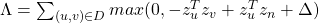where D is a set of training pairs from logs, zv is a “positive”/true example, zn is a “negative” example and Δ is a “margin” (how much larger positive pair similarity should be compared to negative).

3. Inference: Generate embeddings for all pins

4. Nearest neighbour search in embedding space to make recommendations.

Key innovations:

1. On-the-fly graph convolutions:
• Sample the neighboUrhood around a node and dynamically construct a computation graph
• Perform a localized graph convolution around a particular node (At every iteration, only source node embeddings are computed)
• Does not need the entire graph during training
2. Selecting neighbours via random walks
• Performing aggregation on all neighbours is infeasible: how to select the set of neighbours of a node to convolve over? Personalized PageRank can help with that.
• Define Importance pooling: define importance-based neighbourhoods by simulating random walks and selecting the neighbours with the highest visit counts.
• Choose nodes with top K visit counts
• Pool over the chosen nodes
• The chosen nodes are not necessarily neighbours.
• Compared to GraphSAGE mean pooling where the messages are averaged from direct neighbours, PinSAGE Importance pooling use the normalized counts as weights for weighted mean of messages from the top K nodes
• PinSAGE uses ? = 50. Performance gain for ? > 50 is negligible.
3. Efficient MapReduce inference
• Problem: Many repeated computation if using localized graph convolution at inference step.
• Need to avoid repeated computation
1. Introduce hard negative samples: force model to learn subtle distinctions  between pins. Curriculum training on hard negatives starts with random negative examples and then provides harder negative examples over time.  Obtain hard negatives using random walks
• Use nodes with visit counts ranked at 1000-5000 as hard negatives
• Have something in common, but are not too similar
###### Experiments with PinSage

Related Pin recommendations: given a user just saved pin Q, predict what pin X are they going to save next. Setup: Embed 3B pins, find nearest neighbours of Q. Baseline embeddings:

• Visual: VGG visual embeddings.
• Annotation: Word2vec embeddings
• Combined: Concatenate embeddingsPinSage outperform all other models (MRR: Mean reciprocal rank of the positive example X w.r.t Q. Hit rate: Fraction of times the positive example X is among top K closest to Q)

DECAGON: Heterogeneous GNN

So far we only applied GNNs to simple graphs. GNNs do not explicitly use node and edge type  information. Real networks are often heterogeneous. How to use GNN for heterogeneous graphs?

The problem we consider is polypharmacy (use of multiple drugs for a disease): how to predict side effects of drug combination? It is difficult to identify manually because it is rare, occurs only in a subset of patients and is not observed in clinical testing.

Problem formulation:

• How likely with a pair of drugs ?, ? lead to side effect ??
• Graph: Molecules as heterogeneous (multimodal) graphs: graphs with different node types and/or edge types.
• Goal: given a partially observed graph, predict labeled edges between drug nodes. Or in query language: Given a drug pair ?, ? how likely does an edge (? , r2 , ?) exist?

Task description: predict labeled edges between drugs nodes, i.e., predict the likelihood that an edge (? , r2 , s) exists between drug nodes c and s (meaning that drug combination (c, s) leads to polypharmacy side effect r2.

The model is heterogeneous GNN:

• Compute GNN messages from each edge type, then aggregate across different edge types.
• Input: heterogeneous graph.
• Output: node embeddings.
• During edge predictions, use pair of computed node embeddings.
• Input: Node embeddings of query drug pairs.
• Output: predicted edges.

###### Experiment Setup
• Data:
• Graph over Molecules: protein-protein interaction and drug target relationships.
• Graph over Population: Side effects of individual drugs, polypharmacy side effects of drug combinations.
• Setup:
• Construct a heterogeneous graph of all the data .
• Train: Fit a model to predict known associations of drug pairs and polypharmacy side effects.
• Test: Given a query drug pair, predict candidate polypharmacy side effects.

Decagon model showed up to 54% improvement over baselines. It is the first opportunity to computationally flag polypharmacy side effects for follow-up analyses.

##### GCPN: Goal-Directed Graph Generation (an extension of GraphRNN)

Recap Graph Generative Graphs: it generates a graph by generating a two level sequence (uses RNN to generate the sequences of nodes and edges). In other words, it imitates given graphs. But can we do graph generation in a more targeted way and in a more optimal way?

The paper [You et al., NeurIPS 2018] looks into Drug Discovery and poses the question: can we learn a model that can generate valid and realistic molecules with high value of a given chemical property?

Molecules are heterogeneous graphs with node types C, N, O, etc and edge types single bond, double bond, etc. (note: “H”s can be automatically inferred via chemical validity rules, thus are ignored in molecular graphs).

Authors proposes the model Graph Convolutional Policy Network which combines graph representation and Reinforcement Learning (RL):

• Graph Neural Network captures complex structural information, and enables validity check in each state transition (Valid).
• Reinforcement learning optimizes intermediate/final rewards (High scores).
• Adversarial training imitates examples in given datasets (Realistic).

Steps of GCPN and shown on the picture below:

• (a) Insert nodes/scaffolds.
• (b) Compute state via GCN.
• (c) Sample next action.
• (d) Take action (check chemical validity).
• (e, f) Compute reward.

To set the reward in RL part:

• Learn to take valid action: at each step, assign small positive reward for valid action.
• Optimize desired properties: at the end, assign positive reward for high desired property.
• Generate realistic graphs: at the end, adversarially train a GCN discriminator, compute adversarial rewards that encourage realistic molecule graphs

Reward rt is final reward (domain-specific reward) and step reward (step-wise validity reward).

Training consists of two parts:

1. Supervised training: train policy by imitating the action given by real observed graphs. Use gradient.
2. RL training: train policy to optimize rewards. Use standard policy gradient algorithm.

• Property optimization: generate molecules with high specified property score.
• Property targeting: generate molecules whose specified property score falls within given range.
• Constrained property optimization: edit a given molecule for a few steps to achieve higher specified property score.

PS: Other lecture notes you can find here: 12, 3.

## Machine Learning with Graphs: lecture notes, part 3/4

Some time ago, I finished the Stanford course CS224W Machine Learning with Graphs. This is Part 3 of blog posts series where I share my notes from watching lectures. The rest you can find here: 12, 4.

# Lecture 12 – Network Effects and Cascading Behavior

Slides, Video

Information can spread through networks: behaviors that cascade from node to node like an epidemic:

• Diffusion of innovations.
• Network effects.
• Epidemics.

• Biological: diseases via contagion.
• Social: rumors, news, new technology, viral marketing.

Network cascades are contagions that spread over the edges of the network. It creates a propagation tree, i.e., cascade. “Infection” event in this case can be adoption, infection, activation.

Two ways to model model diffusion:

• Decision based models (this lecture ):
• Models of product adoption, decision making.
• A node observes decisions of its neighbors and makes its own decision.
• Example: you join demonstrations if k of your friends do so.
• Probabilistic models (next lecture):
• Models of influence or disease spreading.
• An infected node tries to “push” the contagion to an uninfected node.
• Example: you “catch” a disease with some probability from each active neighbor in the network.
###### Decision based diffusion models

Based on 2 player coordination game: 2 players – each chooses technology A or B; each player can only adopt one “behavior”, A or B; intuition is such that node v gains more payoff if v’s friends have adopted the same behavior as v.

Payoff matrix for model with two nodes looks as following:

• If both v and w adopt behavior A, they each get payoff a > 0.
• If v and w adopt behavior B, they each get payoff b > 0.
• If v and w adopt the opposite behaviors, they each get 0.
• In some large network: each node v is playing a copy of the game with each of its neighbors. Payoff is the sum of node payoffs over all games.

Calculation of node v:

• Let v have d neighbors.
• Assume fraction p of v’s neighbors adopt A.
• Payoffv = a∙p∙d if v chooses A; Payoffv = b∙(1-p)∙d if v chooses B.
• Thus: v chooses A if  p > b / (a+b) = q or  p > q (q is payoff threshold).

Example Scenario:

Assume graph where everyone starts with all B.  Let small set S of early adopters of A be hard-wired set S – they keep using A no matter what payoffs tell them to do. Assume payoffs are set in such a way that nodes say: if more than q = 50% of my friends take A, I’ll also take A. This means: a = b – ε (ε>0, small positive constant) and then q = ½.

###### Application: modeling protest recruitment on social networks

Case: during anti-austerity protests in Spain in May 2011, Twitter was used to organize and mobilize users to participate in the protest. Researchers identified 70 hashtags that were used by the protesters.

• 70 hashtags were crawled for 1 month period. Number of tweets: 581,750.
• Relevant users: any user who tweeted any relevant hashtag and their followers + followees. Number of users: 87,569.
• Created two undirected follower networks:

2. Symmetric network with only the reciprocal follow links (i ➞ j and j ➞ i). This network represents “strong” connections only.

Definitions:

• User activation time: moment when user starts tweeting protest messages.
• kin = the total number of neighbors when a user became active.
• ka = number of active neighbors when a user became active.
• Activation threshold = ka/kin. The fraction of active neighbors at the time when a user becomes active.
• If ka/kin ≈ 0, then the user joins the movement when very few neighbors are active ⇒ no social pressure.
• If ka/kin ≈ 1, then the user joins the movement after most of its neighbors are active ⇒ high social pressure.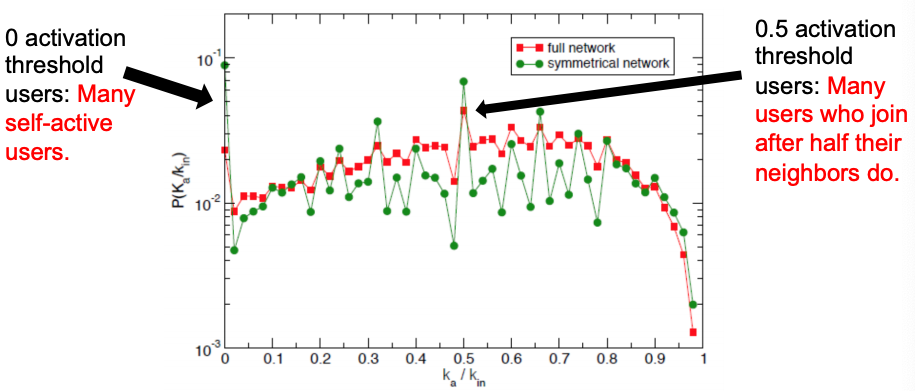Distribution of activation thresholds – mostly uniform distribution in both networks, except for two local peaks.

Authors define the information cascades as follows: if a user tweets a message at time t and one of its followers tweets a message in (t, t + Δt), then they form a cascade.

To identify who starts successful cascades, authors use method of k-core decomposition:

• k-core: biggest connected subgraph where every node has at least degree k.
• Method: repeatedly remove all nodes with degree less than k.
• Higher k-core number of a node means it is more central.

Picture below shows the K-core decomposition of the follow network: red nodes start successful cascades and they have higher k-core values. So, successful cascade starters are central and connected to equally well connected users.

To summarize the cascades on Twitter: users have uniform activation threshold, with two local peaks, most cascades are short, and successful cascades are started by central (more core) users.

So far, we looked at Decision Based Models that are utility based, deterministic and “node” centric (a node observes decisions of its neighbors and makes its own decision – behaviors A and B compete, nodes can only get utility from neighbors of same behavior: A-A get a, B-B get b, A-B get 0). The next model of cascading behavior is the extending decision based models to multiple contagions.

###### Extending the Model: Allow people to adopt A and B

Let’s add an extra strategy “AB”:

• AB-A : gets a.
• AB-B : gets b.
• AB-AB : gets max(a, b).
• Also: some cost c for the effort of maintaining both strategies (summed over all interactions).
• Note: a given node can receive a from one neighbor and b from another by playing AB, which is why it could be worth the cost c.

Cascades and compatibility model: every node in an infinite network starts with B. Then, a finite set S initially adopts A. Run the model for t=1,2,3,…: each node selects behavior that will optimize payoff (given what its neighbors did in at time t-1). How will nodes switch from B to A or AB?

Let’s solve the model in a general case: infinite path starts with all Bs. Payoffs for w: A = a, B = 1, AB = a+1-c. For what pairs (c,a) does A spread? We need to analyze two cases for node w (different neighbors situations) and define what w would do based on the values of a and c?

1. A-w-B: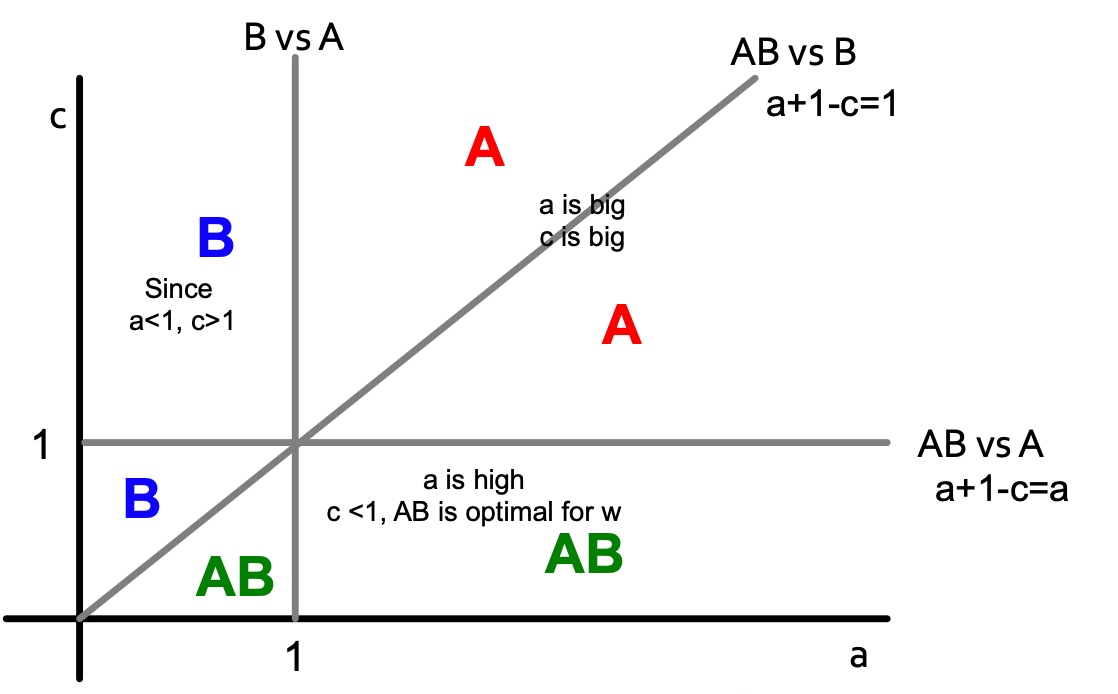Color letters indicate what is the optimal decision for node w (with payoffs for w: A = a, B = 1, AB = a+1-c).

1. AB-w-B: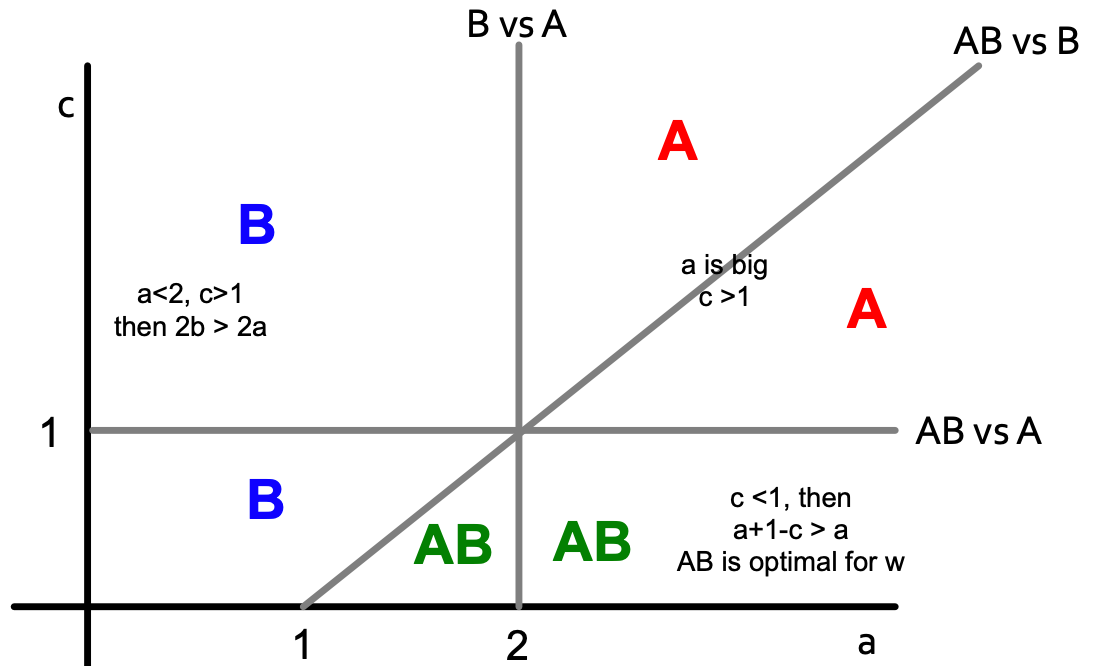Color letters indicate what is the optimal decision for node w (now payoff is changed for B: A = a, B = 1+1, AB = a+1-c).

If we combine two pictures, we get the following:

To summarise: if B is the default throughout the network until new/better A comes along, what happens:

• Infiltration: if B is too compatible then people will take on both and then drop the worse one (B).
• Direct conquest: if A makes itself not compatible – people on the border must choose. They pick the better one (A).
• Buffer zone: if you choose an optimal level then you keep a static “buffer” between A and B.

# Lecture 13 – Probabilistic Contagion and Models of Influence

So far, we learned deterministic decision-based models where nodes make decisions based on pay-off benefits of adopting one strategy or the other. Now, we will do things by observing data because in cascades spreading like epidemics, there is lack of decision making and the process of contagion is complex and unobservable (in some cases it involves (or can be modeled as) randomness).

Simple model: Branching process

• First wave: a person carrying a disease enters the population and transmits to all she meets with probability q. She meets d people, a portion of which will be infected.
• Second wave: each of the d people goes and meets d different people. So we have a second wave of dd = d2 people, a portion of which will be infected.
• Subsequent waves: same process.

Epidemic model based on random trees

• A patient meets d new people and with probability q>0 she infects each of them.
• Epidemic runs forever if: lim (h→∞) p(h) > 0 (p(h) is probability that a node at depth h is infected.
• Epidemic dies out if:  lim (h→∞) p(h) = 0.

So, we need to find  lim (h→∞) p(h) based on q and d. For p(h) to be recurrent (parent-child relation in a tree):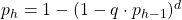Then lim (h→∞) p(h) equals the result of iterating f(x) = 1 – (1 – q ⋅ x)d where x1 = f(1) = 1 (since p1 = 1), x2 = f(x1),  x3 = f(x2), …

If we want the epidemic to die out, then iterating f(x) must go to zero. So, f(x) must be below y = x.

Also, f′(x)  is non-decreasing, and  f′(0) = d ⋅ q, which means that for d ⋅ q > 1, the curve is above the line, and we have a single fixed point of x = 1 (single because f′ is not just monotone, but also non-increasing). Otherwise (if d ⋅ q > 1), we have a single fixed point x = 0. So a simple result: depending on how d ⋅ q compares to 1, epidemic spreads or dies out.

Now we come to the most important number for epidemic R0 = d ⋅ q (d ⋅ q is expected # of people that get infected). There is an epidemic if R0 ≥  1.

Only R0 matters:

• R0 ≥ 1: epidemic never dies and the number of infected people increases exponentially.
• R0  < 1: Epidemic dies out exponentially quickly.

Measures to limit the spreading: When R0 is close 1, slightly changing q or d can result in epidemics dying out or happening:

• Quarantining people/nodes [reducing d].
• Encouraging better sanitary practices reduces germs spreading [reducing q].
• HIV has an R0 between 2 and 5.
• Measles has an R0 between 12 and 18.
• Ebola has an R0 between 1.5 and 2.
###### Application: Social cascades on Flikr and estimating R0 from real data

The paper Characterizing social cascades in Flickr [Cha et al. ACM WOSN 2008] has all details about the application.

Dataset:

• Flickr social network: users are connected to other users via friend links; a user can “like/favorite” a photo.
• Data: 100 days of photo likes; 2 million of users; 34,734,221 likes on 11,267,320 photos.

• Users can be exposed to a photo via social influence (cascade) or external links.
• No, if a user likes a photo and if none of his friends have previously liked the photo.
• Yes, if a user likes a photo after at least one of her friends liked the photo  Social cascade.
• Example social cascade: A B and A C E.

Now let’s estimate R0 from real data. Since R0 = d ⋅ q, need to estimate q first: given an infected node count the proportion of its neighbors subsequently infected and average. Then R0 = q ⋅ d ⋅ (avg(di2)/(avg di )2 where di is degree of node i (last part in the formula is the correction factor due to skewed degree distribution of the network).

Thus, given the start node of a cascade, empirical R0 is the count of the fraction of directly infected nodes.

Authors find that R0 correlates across all photos.

The basic reproduction number of popular photos on Flickr is between 1 and 190. This is much higher than very infectious diseases like measles, indicating that social networks are efficient transmission media and online content can be very infectious.

##### Epidemic  models

[Off-top: the lecture was in November 2019 when COVID-19 just started in China – students were prepared to do analysis of COVID spread in 2020]

Let virus propagation have 2 parameters:

• (Virus) Birth rate β: probability that an infected neighbor attacks.
• (Virus) Death rate δ: probability that an infected node heals.

General scheme for epidemic models: each node can go through several phases and transition probabilities are governed by the model parameters.

###### SIR model
• Node goes through 3 phases: Susceptible -> Infected -> Recovered.
• Models chickenpox or plague: once you heal, you can never get infected again.
• Assuming perfect mixing (the network is a complete graph) the model dynamics are: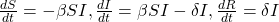where β is transition probability from susceptible to infected phase, δ is transition probability from infected to recovered phase.

###### SIS model
• Susceptible-Infective-Susceptible (SIS) model.
• Cured nodes immediately become susceptible.
• Virus “strength”: s = β  / δ.
• Models flu: susceptible node becomes infected; the node then heals and become susceptible again.
• Assuming perfect mixing (a complete graph):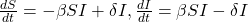Epidemic threshold of an arbitrary graph G with SIS model is τ, such that: if virus “strength” s = β / δ < τ the epidemic can not happen (it eventually dies out).

τ = 1/ λ1,A where λ is the largest eigenvalue of adjacency matrix A of G.

###### SEIR model

Model with phases: Susceptible -> Exposed -> Infected -> Recovered. Ebola outbreak in 2014 is an example of the SEIR model. Paper Gomes et al., 2014 estimates Ebola’s R0 to be 1.5-2.

###### SEIZ model

SEIZ model is an extension of SIS model (Z stands for sceptics).

Paper Jin et al. 2013 applies SEIZ for the modeling of News and Rumors on Twitter. They use tweets from eight stories (four rumors and four real) and fit SEIZ model to data. SEIZ model is fit to each cascade to minimize the difference between the estimated number of rumor tweets by the model and number of rumor tweets: |I(t) – tweets(t)|.

To detect rumors, the use new metric (parameters are the same as on figure above):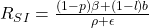RSI is a kind of flux ratio, the ratio of effects entering E to those leaving E.

All parameters learned by model fitting to real data.

Initially some nodes S are active. Each edge (u,v) has probability (weight) puv. When node u becomes active/infected, it activates each out-neighbor v with probability puv. Activations spread through the network.

Independent cascade model is simple but requires many parameters. Estimating them from data is very hard [Goyal et al. 2010]. The simple solution is to make all edges have the same weight (which brings us back to the SIR model). But it is too simple. We can do something better with exposure curves.

• Exposure: node’s neighbor exposes the node to the contagion.
• Adoption: the node acts on the contagion.

Probability of adopting new behavior depends on the total number of friends who have already adopted. Exposure curves show this dependence.

Exposure curves are used to show diffusion in viral marketing, e.g. when senders and followers of recommendations receive discounts on products (what is the probability of purchasing given number of recommendations received?) or group memberships spread over the social network (How does probability of joining a group depend on the number of friends already in the group?).

Parameters of the exposure curves:

• Persistence of P is the ratio of the area under the curve P and the area of the rectangle of height max(P), width max(D(P)).
• D(P) is the domain of P.
• Persistence measures the decay of exposure curves.
• Stickiness of P is max(P): the probability of usage at the most effective exposure.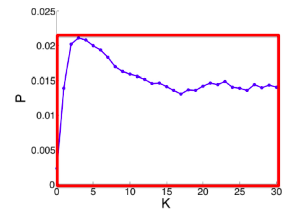Persistence of P is the ratio of the area under the blue curve P and the area of the red rectangle

Paper Romero et al. 2011 studies exposure curve parameters for twitter data. They find that:

• Idioms and Music have lower persistence than that of a random subset of hashtags of the same size.
• Politics and Sports have higher persistence than that of a random subset of hashtags of the same size.
• Technology and Movies have lower stickiness than that of a random subset of hashtags. Music has higher stickiness than that of a random subset of hashtags (of the same size).

# Lecture 14 – Influence Maximization in Networks

Viral marketing is based on the fact that we are more influenced by our friends than strangers. For marketing to be viral, it identifies influential customers, convinces them to adopt the product  (offer discount or free samples) and then these customers endorse the product among their friends.

Influence maximisation is a process (given a directed graph and k>0) of finding k seeds to maximize the number of influenced people (possibly in many steps).

There exists two classical propagation models: linear threshold model and independent cascade model.

###### Linear threshold model
• A node v has a random threshold θv ~ U[0.1].
• A node v is influenced by each neighbor w according to a weight bv,w such that Σbv,w 1.
• A node v becomes active when at least (weighted) θv fraction of its neighbors are active: Σbv,w 0.
###### Probabilistic Contagion – Independent cascade model
• Directed finite G = (V, E).
• Set S starts out with new behavior. Say nodes with this behavior are “active”.
• Each edge (v,w) has a probability pvw.
• If node v is active, it gets one chance to make w active, with probability pvw. Each edge fires at most once. Activations spread through the network.
• Scheduling doesn’t matter. If u, v are both active at the same time, it doesn’t matter which tries to activate w first. But the time moves in discrete steps.

Most influential set of size k: set S of k nodes producing largest expected cascade size f(S) if activated. It translates to optimization problem max f(S). Set S is more influential if f(S) is larger.

###### Approximation algorithm for influence maximization

Influence maximisation is NP-complete (the optimisation problem is max(over S of size k) f(S) to find the most influential set S on k nodes producing largest expected cascade size f(S) if activated). But there exists an approximation algorithm:

• For some inputs the algorithm won’t find a globally optimal solution/set OPT.
• But we will also prove that the algorithm will never do too badly either. More precisely, the algorithm will find a set S that where f(S) ≥ 0.63*f(OPT), where OPT is the globally optimal set.

Consider a Greedy Hill Climbing algorithm to find S.  Input is the influence set Xu of each node u: Xu = {v1, v2, … }. That is, if we activate u, nodes {v1, v2, … } will eventually get active.The algorithm is as following: at each iteration i activate the node u that gives largest marginal gain: max (over u) f(Si-1 ∪ {u}).

For example on the picture above:

• Evaluate f({a}) , … , f({e}), pick argmax of them.
• Evaluate f({d,a}) , … , f({d,e}), pick argmax.
• Evaluate f({d,b,a}) , … , f({d,b,e}), pick argmax.

Hill climbing produces a solution S where: f(S) ≥ (1-1/e)*f(OPT) or (f(S)≥ 0.63*f(OPT)) (referenced to Nemhauser, Fisher, Wolsey ’78, Kempe, Kleinberg, Tardos ‘03). This claim holds for functions f(·) with 2 properties (lecture contains proves for both properties, I omit them here):

1. f is monotone: (activating more nodes doesn’t hurt) if S ⊆ T then f(S) ≤ f(T) and f({}) = 0.
2. f is submodular (activating each additional node helps less): adding an element to a set gives less improvement than adding it to one of its subsets:   ∀ S ⊆  T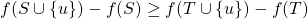where the left-hand side is a gain of adding a node to a small set, the right-hand side is a gain of adding a node to a large set.

The bound (f(S)≥ 0.63 * f(OPT)) is data independent. No matter what is the input data, we know that the Hill-Climbing will never do worse than 0.63*f(OPT).

Evaluate of influence maximization ƒ(S) is still an open question of how to compute it efficiently. But there are very good estimates by simulation: repeating the diffusion process often enough (polynomial in n; 1/ε). It achieves (1± ε)-approximation to f(S).

Greedy approach is slow:

• For a given network G, repeat 10,000s of times:
• flip the coin for each edge and determine influence sets under coin-flip realization.
• each node u is associated with 10,000s influence sets Xui .
• Greedy’s complexity is O(k ⋅ n ⋅ R ⋅ m) where n is the number of nodes in Gk is the number of nodes to be selected/influenced, R is the number of simulation rounds (number possible worlds), m is the number of edges in G.
###### Experiment data
• A collaboration network: co-authorships in papers of the arXiv high-energy physics theory: 10,748 nodes, 53,000 edges. Example of a cascade process: spread of new scientific terminology/method or new research area.
• Independent Cascade Model: each user’s threshold is uniform random on [0,1].
• Case 1: uniform probability p on each edge.
• Case 2: Edge from v to w has probability 1/deg(w) of activating w.
• Simulate the process 10,000 times for each targeted set. Every time re-choosing edge outcomes randomly.
• Compare with other 3 common heuristics:
• Degree centrality: pick nodes with highest degree.
• Closeness centrality: pick nodes in the “center” of the network.
• Random nodes: pick a random set of nodes.
• Greedy algorithm outperforms other heuristics (as shown on pictures below).

##### Speeding things up: sketch-based algorithms

Recap that to perform influence maximization we need to generate a number R of possible worlds and then identify k nodes with the largest influence in these possible worlds. To solve the problem that for any given node set, evaluating its influence in a possible world takes O(m) time (m is the number of edges), we will use sketches to reduce estimation time from O(m) to O(1).

Idea behind sketches:

• Compute small structure per node from which to estimate its influence. Then run influence maximization using these estimates.
• Take a possible world G(i). Give each node a uniform random number from [0,1]. Compute the rank of each node v, which is the minimum number among the nodes that v can reach.
• Intuition: if v can reach a large number of nodes then its rank is likely to be small. Hence, the rank of node v can be used to estimate the influence of node v a graph in a possible word  G(i).

Sketches have a problem: influence estimation based on a single rank/number can be inaccurate:

• One solution is to keep multiple ranks/numbers, e.g., keep the smallest c values among the nodes that v can reach. It enables an estimate on union of these reachable sets.
• Another solution is to keep multiple ranks (say c of them): keep the smallest c values among the nodes that v can reach in all possible worlds considered (but keep the numbers fixed across the worlds).
###### Sketch-based Greedy algorithm

Steps of the algorithm:

• Generate a number of possible worlds.
• Construct reachability sketches for all node:
• Result: each node has c ranks.
• Run Greedy for influence maximization:
• Whenever Greedy asks for the influence of a node set S, check ranks and add a u node that has the smallest value (lexicographically).
• After u is chosen, find its influence set of nodes f(u), mark them as infected and remove their “numbers” from the sketches of other nodes.

Guarantees:

• Expected running time is near-linear in the number of possible worlds.
• When c is large, it provides (1 − 1 / ε  − ε) approximation with respect to the possible worlds considered.

• Expected near-linear running time.
• Provides an approximation guarantee with respect to the possible worlds considered.

• Does not provide an approximation guarantee on the ”true” expected influence.

# Lecture 15 – Outbreak Detection in Networks

The problem this lecture discusses: given a dynamic process spreading over a network we want to select a set of nodes to detect the process effectively. There are many applications:

• Epidemics (given a real city water distribution network and data on how contaminants spread in the network detect the contaminant as quickly as possible).
• Influence propagation (which users/news sites should one follow to detect cascades as effectively as possible?).
• Network security.

Problem setting for Contamination problem: given graph G(V, E), data about how outbreaks spread over the for each outbreak i we know the time T(u,i) when outbreak i contaminates node u. The goal is to select a subset of nodes S that maximizes the expected reward for detecting outbreak i: max f(S) = Σ p(i) fi(S) subject to cost(S) < B where p(i) is the probability of outbreak i occurring, f(i) is the reward for detecting outbreak i using sensors S.

Generally, the problem has two parts:

1. Reward (one of the following three):
1. Minimize time to detection.
2. Maximize number of detected propagations.
3. Minimize the number of infected people.
2. Cost (context dependent):
1. Reading big blogs is more time consuming.
2. Placing a sensor in a remote location is expensive.

Let set a penalty πi(t) for detecting outbreak i at time t. Then for all three reward settings  detecting sooner does not hurt:

1. Time to detection (DT): how long does it take to detect a contamination?
• Penalty for detecting at time t: πi(t) = t.
2. Detection likelihood (DL): how many contaminations do we detect?
• Penalty for detecting at time t: πi(t) = 0, πi(∞) = 1. Note, this is a binary outcome: we either detect or not.
3. Population affected (PA): how many people drank contaminated water?
• Penalty for detecting at time t: πi(t) = {# of infected nodes in outbreak i by time t}.

Now, let’s define fi(S) as penalty reduction: fi(S) = πi(∞) − πi(T(S, i)). With this we can observe diminishing returns:

Now we can see that objective function is submodular (recall from previous lecture:  f is submodular if activating each additional node helps less).

What do we know about optimizing submodular functions?

• Hill-climbing (i.e., greedy) is near optimal: (1 − 1 / e ) ⋅ OPT.
• But this only works for unit cost case (each sensor costs the same):
• For us each sensor s has cost c(s).
• Hill-climbing algorithm is slow: at each iteration we need to re-evaluate marginal gains of all nodes.
• Runtime O(|V| · K) for placing K sensors.
###### Towards a new algorithm

Consider the following algorithm to solve the outbreak detection problem: Hill-climbing that ignores cost:

• Ignore sensor cost c(s).
• Repeatedly select sensor with highest marginal gain.
• Do this until the budget is exhausted.

But it can fail arbitrarily badly. There exists a problem setting where the hill-climbing solution is arbitrarily far from OPT. Next we come up with an example.

Bad example when we ignore cost:

• n sensors, budget B.
• s1: reward r, cost c.
• s2…sn: reward r − ε, c = 1.
• Hill-climbing always prefers more expensive sensor s1 with reward r (and exhausts the budget). It never selects cheaper sensors with reward r − ε → For variable cost it can fail arbitrarily badly.

Bad example when we optimize benefit-cost ratio (greedily pick sensor si that maximizes benefit to cost ratio):

• Budget B.
• 2 sensors s1 and s2: costs c(s1) = ε, c(s1) = B; benefit (only 1 cascade): f(s1) = 2ε, f(s2) = B.
• Then the benefit-cost ratio is: f(s1) / c(s1) = 2 and f(s2) / c(s2) = 1. So, we first select s1 and then can not afford s2  → We get reward 2ε instead of B. Now send ε → 0 and we get an arbitrarily bad solution.
• This algorithm incentivizes choosing nodes with very low cost, even when slightly more expensive ones can lead to much better global results.

The solution is the CELF (Cost-Effective Lazy Forward-selection) algorithm. It has two passes: set (solution) S′ – use benefit-cost greedy and Set (solution) S′′ – use unit-cost greedy. Final solution: S = arg max ( f(S’), f(S”))

CELF is near optimal [Krause&Guestrin, ‘05]: it achieves ½(1-1/e) factor approximation. This is surprising: we have two clearly suboptimal solutions, but taking best of the two is guaranteed to give a near-optimal solution.

###### Speeding-up Hill-Climbing: Lazy Evaluations

Idea: use δi as upper-bound on δj (j > i). Then for lazy hill-climbing keep an ordered list of marginal benefits δi from the previous iteration and re-evaluate δi only for top node, then re-order and prune.

CELF (using Lazy evaluation) runs 700 times faster than greedy hill-climbing algorithm:

###### Data Dependent Bound on the Solution Quality

The (1-1/e) bound for submodular functions is the worst case bound (worst over all possible inputs). Data dependent bound is a value of the bound dependent on the input data. On “easy” data, hill climbing may do better than 63%. Can we say something about the solution quality when we know the input data?

Suppose S is some solution to f(S) s.t. |S| ≤ k ( f(S) is monotone & submodular):

• Let OPT = {ti, … , tk} be the OPT solution.
• For each u let δ(u) = f(S ∪ {u} − f(S).
• Order δ(u) so that δ(1)δ(2) ≥ ⋯
• Then: f(OPT) ≤ f(S) + ∑δ(i)
• Note: this is a data dependent bound (δ(i) depends on input data). Bound holds for any algorithm. It makes no assumption about how S was computed. For some inputs it can be very “loose” (worse than 63%).
###### Case Study: Water Network

Real metropolitan area water network with V = 21,000 nodes and E = 25,000 pipes. Authors [Ostfeld et al., J. of Water Resource Planning] used a cluster of 50 machines for a month to simulate 3.6 million epidemic scenarios (random locations, random days, random time of the day).

Main results:

• Data-dependent bound is much tighter (gives more accurate estimate of algorithmic performance).
• Placement heuristics perform much worse.
• CELF is much faster than greedy hill-climbing (but there might be datasets/inputs where the CELF will have the same running time as greedy hill-climbing):

• Different objective functions give different sensor placements:

###### Case Study: Cascades in blogs

Setup:

• Crawled 45,000 blogs for 1 year.
• Obtained 10 million news posts and identified 350,000 cascades.
• Cost of a blog is the number of posts it has.

Main results:

• Online bound turns out to be much tighter: 87% instead of 32.5%.
• Heuristics perform much worse: one really needs to perform the optimization.
• CELF runs 700 times faster than a simple hill-climbing algorithm.

CELF has 2 sub-algorithms:

• Unit cost: CELF picks large popular blogs.
• Cost-benefit: cost proportional to the number of posts.
• We can do much better when considering costs.
• But there is a problem: CELF picks lots of small blogs that participate in few cascades. Thus, we pick best solution that interpolates between the costs -> we can get good solutions with few blogs and few posts.

We want to generalize well to future (unknown) cascades. Limiting selection to bigger blogs improves generalization:

# Lecture 16 – Network Evolution

Evolving networks are networks that change as a function of time. Almost all real world networks evolve either by adding or removing nodes or links over time. Examples are social networks (people make and lose friends and join or leave the network), internet, web graphs, e-mail, phone calls, P2P networks, etc.

The picture below shows the largest components in Apple’s inventor network over a 6-year period. Each node reflects an inventor, each tie reflects a patent collaboration. Node colors reflect technology classes, while node sizes show the overall connectedness of an inventor by measuring their total number of ties/collaborations (the node’s so-called degree centrality).

There are three levels of studying evolving networks:

1. Macro level (evolving network models, densification).
2. Meso level (Network motifs, communities).
3. Micro level (Node, link properties – degree, network centrality).
###### Macroscopic evolution of networks

The questions we answer in this part are:

1. What is the relation between the number of nodes n(t) and number of edges e(t) over time t?
2. How does diameter change as the network grows?
3. How does degree distribution evolve as the network grows?

Q1: Let’s set at time t nodes N(t), edges E(t) and suppose that N(t+1) = 2 ⋅ N(t). What is now E(t+1)? It is more than doubled. Networks become denser over time obeying Densification Power Law: E(t) ∝ N(t)a where a is densification exponent (1 ≤ a ≤ 2). In other words, it shows that the number of edges grows faster than the number of nodes – average degree is increasing. When a=1, the growth is linear with constant out-degree (traditionally assumed), when a=2, the growth is quadratic – the graph is fully connected.

Q2: As the network grows the distances between the nodes slowly decrease, thus diameter shrinks over time (recap how we compute diameter in practice: with long paths, take 90th-percentile or average path length (not the maximum); with disconnected components, take only the largest component or average only over connected pairs of nodes).

But consider densifying random graph: it has increasing diameter (on picture below). There is more to shrinking diameter than just densification.

Comparing rewired random network to real network (all with the same degree distribution) shows that densification and degree sequence gives shrinking diameter.

But how to model graphs that densify and have shrinking diameters? (Intuition behind this: How do we meet friends at a party? How do we identify references when writing papers?)

###### Forest Fire Model

The Forest Fire model has 2 parameters: p is forward burning probability,  r is backward burning probability. The model is Directed Graph. Each turn a new node v arrives. And then:

1. v chooses an ambassador node w uniformly at random, and forms a link to w.
2. Flip 2 coins sampled from a geometric distribution: generate two random numbers x and y from geometric distributions with means p / (1 − p) and rp / (1 − rp).
3. v selects x out-links and y in-links of w incident to nodes that were not yet visited and form out-links to them (to ”spread the fire” along).
4. v applies step (2) to the nodes found in step (3) (“Fire” spreads recursively until it dies; new node v links to all burned nodes).

On the picture above:

1. Connect to a random node w.
2. Sample x = 2, y = 1.
3. Connect to 2 out- and 1 in-links of w, namely a,b,c.
4. Repeat the process for a,b,c.

In the described way, Forest Fire generates graphs that densify and have shrinking diameter:

Also, Forest Fire generates graphs with power-law degree distribution:

We can fix backward probability r and vary forward burning probability p. Notice a sharp transition between sparse and clique-like graphs on the plot below. The “sweet spot” is very narrow.

###### Temporal Networks

Temporal network is a sequence of static directed graphs over the same (static) set of nodes V. Each temporal edge is a timestamped ordered pair of nodes (ei = (u, v) , ti), where u, v ∈ V and ti is the timestamp at which the edge exists, meaning that edges of a temporal network are active only at certain points in time.

Temporal network examples:

• Communication: Email, phone call, face-to-face.
• Proximity networks: Same hospital room, meet at conference, animals hanging out.
• Transportation: train, flights.
• Cell biology: protein-protein, gene regulation.
###### Microscopic evolution of networks

The questions we answer in this part are:

• How do we define paths and walks in temporal networks?
• How can we extend network centrality measures to temporal networks?
###### Q1: Path

A temporal path is a sequence of edges (u1, u2, t1), (u2, u3, t2), … , (uj, uj+1, tj) for which t1t2 ≤ ⋯ ≤ tj and each node is visited at most once.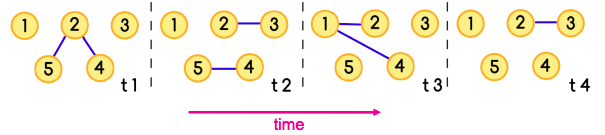The sequence of edges [(5,2),(2,1)] together with the sequence of times t1, t3 is a temporal path

To find the temporal shortest path, we use the TPSP-Dijkstra algorithm – an adaptation of Dijkstra using a priority queue. Briefly, it includes the following steps:

• Set distance to ∞ for all nodes.
• Set distance to 0 for ns (source node).
• Insert (nodes, distances) to PQ (priority queue).
• Extract the closest node from PQ.
• Verify if edge e is valid at tq (time of the query – we calculate the distance from source node ns to target node nt between time ts and time tq).
• If so, update v’s distance from ns.
• insert (v, d[v]) to PQ or update d[v] in PQ where d[v] is the distance of ns to v.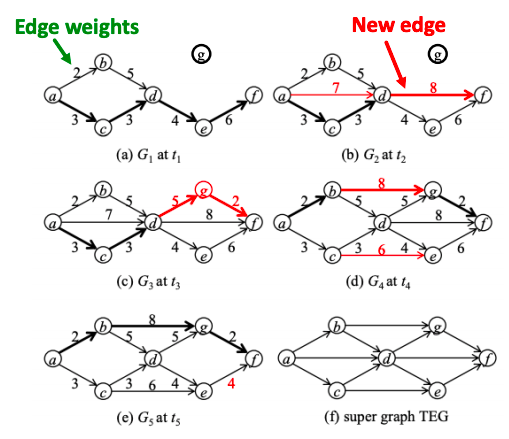Example of a temporally evolving graph. Shortest paths from a to f are marked in thick lines.

###### Q2: Centrality

Temporal closeness is the measure of how close a node is to any other node in the network at time interval [0,t]. Sum of shortest (fastest) temporal path lengths to all other nodes is: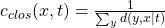where denominator is the length of the temporal shortest path from y to x from time 0 to time t.

Temporal PageRank idea is to make a random walk only on temporal or time-respecting paths.

• A temporal or time-respecting walk is a sequence of edges (u1, u2, t1), (u2, u3, t2), … , (uj, uj+1, tj) for which t1t2 ≤ ⋯ ≤ tj.

As t → ∞, the temporal PageRank converges to the static PageRank. Explanation is this:

• Temporal PageRank is running regular PageRank on a time augmented graph:
• Connect graphs at different time steps via time hops, and run PageRank on this time-extended graph.
• Node u at ti becomes a node (u,  ti) in this new graph.
• Transition probabilities given by P ((u, ti ), (x, t2 )) | (v, t0, (u, ti ) = β| Гu | .
• As t → ∞, β| Гu |  becomes the uniform distribution: graph looks as if we superimposed the original graphs from each time step and we are back to regular PageRank.

How to compute temporal PageRank:

• Initiating a new walk with probability 1 − α.
• With probability α we continue active walks that wait in u.
• Increment the active walks (active mass) count in the node v with appropriate normalization 1−β.
• Decrements the active mass count in node u.
• Increments the active walks (active mass) count in the node v with appropriate normalization 1−β.
• Decrements the active mass count in node u.

In math words, temporal PageRank is: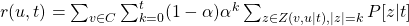where Z(v,u | t ) is a set of all possible temporal walks from v to u until time t and α is the probability of starting a new walk.

Case Studies for temporal PageRank:

• Facebook: A 3-month subset of Facebook activity in a New Orleans regional community. The dataset contains an anonymized list of wall posts (interactions).
• Twitter: Users’ activity in Helsinki during 08.2010– 10.2010. As interactions we consider tweets that contain mentions of other users.
• Students: An activity log of a student online community at the University of California, Irvine. Nodes represent students and edges represent messages.

Experimental setup:

• For each network, a static subgraph of n = 100 nodes is obtained by BFS from a random node.
• Edge weights are equal to the frequency of corresponding interactions and are normalized to sum to 1.
• Then a sequence of 100K temporal edges are sampled, such that each edge is sampled with probability proportional to its weight.
• In this setting, temporal PageRank is expected to converge to the static PageRank of a corresponding graph.
• Probability of starting a new walk is set to α = 0.85, and transition probability β for temporal PageRank is set to 0 unless specified otherwise.

Results show that rank correlation between static and temporal PageRank is high for top-ranked nodes and decreases towards the tail of ranking:

Another finding is that smaller β corresponds to slower convergence rate, but better correlated rankings:

###### Mesoscopic evolution of networks

The questions we answer in this part are:

• How do patterns of interaction change over time?
• What can we infer about the network from the changes in temporal patterns?
###### Q1: Temporal motifs

k−node l−edge δ-temporal motif is a sequence of l edges (u1,v1, t1), (u2,v2, t2), …, (ul,vl, tl) such that t1 < t2 < … < tl and tlt1δ. The induced static graph from the edges is connected and has k nodes.

Temporal motifs offer valuable information about the networks’ evolution: for example, to discover trends and anomalies in temporal networks.

δ-temporal Motif Instance is a collection of edges in a temporal graph if it matches the same edge pattern, and  all of the edges occur in the right order specified by the motif, within a δ time window.

###### Q2: Case Study – Identifying trends and anomalies

Consider all 2- and 3- node motifs with 3 edges: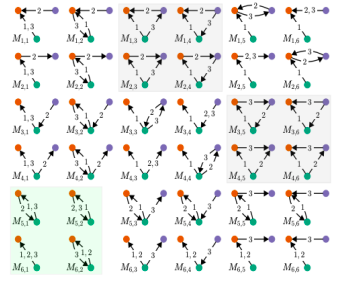The green background highlights the four 2-node motifs (bottom left) and the grey background highlights the eight triangles.

The study [Paranjape et al. 2017] looks at 10 real-world temporal datasets. Main results are:

• Blocking communication (if an individual typically waits for a reply from one individual before proceeding to communicate with another individual): motifs on the left on the picture below capture “blocking” behavior, common in SMS messaging and Facebook wall posting, and motifs on the right exhibit “non-blocking” behavior, common in email.

• Cost of Switching:
• On Stack Overflow and Wikipedia talk pages, there is a high cost to switch targets because of peer engagement and depth of discussion.
• In the COLLEGEMSG dataset there is a lesser cost to switch because it lacks depth of discussion within the time frame of δ = 1 hour.
• In EMAIL-EU, there is almost no peer engagement and cost of switching is negligible

###### Case Study – Financial Network

To spot trends and anomalies, we have to spot statistically significant temporal motifs. To do so, we must compute the expected number of occurrences of each motif:

• Data: European country’s transaction log for all transactions larger than 50K Euros over 10 years from 2008 to 2018, with 118,739 nodes and 2,982,049 temporal edges (δ=90 days).
• Anomalies: we can localize the time the financial crisis hits the country around September 2011 from the difference in the actual vs. expected motif frequencies.

PS: Other lecture notes you can find here: 12, 4.

## Machine Learning with Graphs: lecture notes, part 2/4

Recently, I finished the Stanford course CS224W Machine Learning with Graphs. This is Part 2 of blog posts series where I share my notes from watching lectures. The rest you can find here: 1, 3, 4.

# Lecture 6 – Message Passing and Node Classification

Node classification means: given a network with labels on some nodes, assign labels to all other nodes in the network. The main idea for this lecture is to look at collective classification leveraging existing correlations in networks.

Individual behaviors are correlated in a network environment. For example, the network where nodes are people, edges are friendship, and the color of nodes is a race: people are segregated by race due to homophily  (the tendency of individuals to associate and bond with similar others).

“Guilt-by-association” principle: similar nodes are typically close together or directly connected; if I am connected to a node with label X, then I am likely to have label X as well.

###### Collective classification

Intuition behind this method: simultaneously classify interlinked nodes using correlations.

Applications of the method are found in:

• document classification,
• speech tagging,
• optical character recognition,
• image/3D data segmentation,
• entity resolution in sensor networks,
• spam and fraud detection.

Collective classification is based on Markov Assumption – the label Yi of one node i depends on the labels of its neighbours Ni: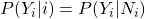Steps of collective classification algorithms:

1. Local Classifier: used for initial label assignment.
• Predict label based on node attributes/features.
• Don’t use network information.
2. Relational Classifier: capture correlations between nodes based on the network.
• Learn a classifier to label one node based on the labels and/or attributes of its neighbors.
• Network information is used.
3. Collective Inference: propagate the correlation through the network.
• Apply relational classifier to each node iteratively.
• Iterate until the inconsistency between neighboring labels is minimized.
• Network structure substantially affects the final prediction.

If we represent every node as a discrete random variable with a joint mass function p of its class membership, the marginal distribution of a node is the summation of p over all the other nodes. The exact solution takes exponential time in the number of nodes, therefore they use inference techniques that approximate the solution by narrowing the scope of the propagation (e.g., only neighbors) and the number of variables by means of aggregation.

Techniques for approximate inference (all are iterative algorithms):

• Relational classifiers (weighted average of neighborhood properties, cannot take node attributes while labeling).
• Iterative classification (update each node’s label using own and neighbor’s labels, can consider node attributes while labeling).
• Belief propagation (Message passing to update each node’s belief of itself based on neighbors’ beliefs).
###### Probabilistic Relational classifier

The idea is that class probability of Yi  is a weighted average of class probabilities of its neighbors.

• For labeled nodes, initialize with ground-truth Y labels.
• For unlabeled nodes, initialize Y uniformly.
• Update all nodes in a random order until convergence or until maximum number of iterations is reached.

Repeat for each node i and label c: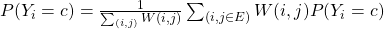W(i,j) is the edge strength from i to j.

Example of 1st iteration for one node:

Challenges:

• Convergence is not guaranteed.
• Model cannot use node feature information.
###### Iterative classification

Main idea of iterative classification is to classify node i based on its attributes as well as labels of neighbour set Ni:

Architecture of iterative classifier:

• Bootstrap phase:
• Convert each node i to a flat vector ai (Node may have various numbers of neighbours, so we can aggregate using: count , mode, proportion, mean, exists, etc.)
• Use local classifier f(ai) (e.g., SVM, kNN, …) to compute best value for Yi .
• Iteration phase: iterate till convergence. Repeat for each node i:
• Update node vector ai.
• Update label Yi to f(ai)
• Iterate until class labels stabilize or max number of iterations is reached.

Note: Convergence is not guaranteed (need to run for max number of iterations).

Application of iterative classification:

• REV2: Fraudulent User Predictions in Rating Platforms [Kumar et al.]. The model uses fairness, goodness and reliability scores to find malicious apps and users who give fake ratings.
###### Loopy belief propagation

Belief Propagation is a dynamic programming approach to answering conditional probability queries in a graphical model. It is an iterative process in which neighbor variables “talk” to each other, passing messages.

In a nutshell, it works as follows: variable X1 believes variable X2 belongs in these states with various likelihood. When consensus is reached, calculate final belief.

Message passing:

• Task: Count the number of nodes in a graph.
• Condition: Each node can only interact (pass message) with its neighbors.
• Solution: Each node listens to the message from its neighbor, updates it, and passes it forward.

Loopy belief propagation algorithm:

Initialize all messages to 1. Then repeat for each node: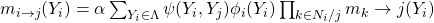• Label-label potential matrix ψ: dependency between a node and its neighbour. ψ(Yi , Yj) equals the probability of a node j being in state Yj  given that it has a i neighbour in state Yi .
• Prior belief ϕi: probability ϕi(Yi) of node i being in state Yi.
• m(i->j)(Yi) is i’s estimate of j being in state Yj.
• Λ is the set of all states.
• Last term (Product) means that all messages sent by neighbours from previous round.
• After convergence bi(Yi) = i’s belief of being in state Yi: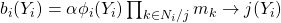• Advantages of the method: easy to program & parallelize, can apply to any graphical model with any form of potentials (higher order than pairwise).
• Challenges: convergence is not guaranteed (when to stop), especially if many closed loops.
• Potential functions (parameters): require training to estimate, learning by gradient-based optimization (convergence issues during training).

Application of belief propagation:

• Netprobe: A Fast and Scalable System for Fraud Detection in Online Auction Networks [Pandit et al.]. Check out mode details here. It describes quite interesting case when fraudsters form a buffer level of “accomplice” users to game the feedback system.

# Lecture 7 – Graph Representation Learning

Supervised Machine learning algorithm includes feature engineering. For graph ML, feature engineering is substituted by feature representation – embeddings. During network embedding, they map each node in a network into a low-dimensional space:

• It gives distributed representations for nodes;
• Similarity of embeddings between nodes indicates their network similarity;
• Network information is encoded into generated node representation.

Network embedding is hard because graphs have complex topographical structure (i.e., no spatial locality like grids), no fixed node ordering or reference point (i.e., the isomorphism problem) and they often are dynamic and have multimodal features.

###### Embedding Nodes

Setup: graph G with vertex set V, adjacency matrix A (for now no node features or extra information is used).

Goal is to encode nodes so that similarity in the embedding space (e.g., dot product) approximates similarity in the original network.

Learning Node embeddings:

1. Define an encoder (i.e., a mapping from nodes to embeddings).
2. Define a node similarity function (i.e., a measure of similarity in the original network).

Optimize the parameters of the encoder so that: Similarity (u, v) ≈ zTvzu.

Two components:

• Encoder: maps each node to a low dimensional vector. Enc(v) = zv where v is node in the input graph and zv  is d-dimensional embedding.
• Similarity function: specifies how the relationships in vector space map to the relationships in the original network – zTvzu is a dot product between node embeddings.
###### “Shallow” encoding

The simplest encoding approach is when encoder is just an embedding-lookup.

Enc(v) = Zv where Z is a matrix with each column being a node embedding (what we learn) and v is an indicator vector with all zeroes except one in column indicating node v.

Many methods for encoding: DeepWalk, node2vec, TransE. The methods are different in how they define node similarity (should two nodes have similar embeddings if they are connected or share neighbors or have similar “structural roles”?).

###### Random Walk approaches to node embeddings

Given a graph and a starting point, we select a neighbor of it at random, and move to this neighbor; then we select a neighbor of this point at random, and move to it, etc. The (random) sequence of points selected this way is a random walk on the graph.

Then zTvzu probability that u and v co-occur on a random walk over the network.

Properties of random walks:

• Expressivity: Flexible stochastic definition of node similarity that incorporates both local and higher-order neighbourhood information.
• Efficiency: Do not need to consider all node pairs when training; only need to consider pairs that co-occur on random walks.

Algorithm:

1. Run short fixed-length random walks starting from each node on the graph using some strategy R.
2. For each node u collect NR(u), the multiset (nodes can repeat) of nodes visited on random walks starting from u.

Optimize embeddings: given node u, predict its neighbours NR(u)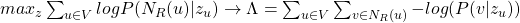Intuition: Optimize embeddings to maximize likelihood of random walk co-occurrences.

Then parameterize it using softmax: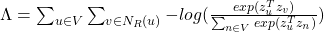where first sum is sum over all nodes u, second sum is sum over nodes v seen on random walks starting from u and fraction (under log) is predicted probability of u and v co-occuring on random walk.

Optimizing random walk embeddings = Finding embeddings zu that minimize Λ. Naive optimization is too expensive, use negative sampling to approximate.

Strategies for random walk itself:

• Simplest idea: just run fixed-length, unbiased random walks starting from each node (i.e., DeepWalk from Perozzi et al., 2013). But such notion of similarity is too constrained.
• More general: node2vec.
###### Node2vec: biased walks

Idea: use flexible, biased random walks that can trade off between local and global views of the network (Grover and Leskovec, 2016).

Two parameters:

1. Return parameter p: return back to the previous node
2. In-out parameter q: moving outwards (DFS) vs. inwards (BFS). Intuitively, q is the “ratio” of BFS vs. DFS

Node2vec algorithm:

1. Compute random walk probabilities.
2. Simulate r random walks of length l starting from each node u.
3. Optimize the node2vec objective using Stochastic Gradient Descent.

How to use embeddings zi of nodes:

• Clustering/community detection: Cluster points zi.
• Node classification: Predict label f(zi) of node i based on zi.
• Link prediction: Predict edge (i,j) based on f(zi,zj) with concatenation, avg, product, or the difference between the embeddings.

Random walk approaches are generally more efficient.

###### Translating embeddings for modeling multi-relational data

A knowledge graph is composed of facts/statements about inter-related entities. Nodes are referred to as entities, edges as relations. In Knowledge graphs (KGs), edges can be of many types!

Knowledge graphs may have missing relation. Intuition: we want a link prediction model that learns from local and global connectivity patterns in the KG, taking into account entities and relationships of different types at the same time.

Downstream task: relation predictions are performed by using the learned patterns to generalize observed relationships between an entity of interest and all the other entities.

In Translating Embeddings method (TransE), relationships between entities are represented as triplets h (head entity), l (relation), t (tail entity) => (ℎ, l, t).

Entities are first embedded in an entity space Rk and relations are represented as translations  ℎ + l ≈ t if the given fact is true, else, ℎ + lt.

###### Embedding entire graphs

Sometimes we need to embed entire graphs to such tasks as classifying toxic vs. non-toxic molecules or identifying anomalous graphs.

Approach 1:

Run a standard graph embedding technique on the (sub)graph G. Then just sum (or average) the node embeddings in the (sub)graph G. (Duvenaud et al., 2016-  classifying molecules based on their graph structure).

Approach 2:

Idea: Introduce a “virtual node” to represent the (sub)graph and run a standard graph embedding technique (proposed by Li et al., 2016 as a general technique for subgraph embedding).

Approach 3 – Anonymous Walk Embeddings:

States in anonymous walk correspond to the index of the first time we visited the node in a random walk.

Anonymous Walk Embeddings have 3 methods:

1. Represent the graph via the distribution over all the anonymous walks (enumerate all possible anonymous walks ai of l steps, record their counts and translate it to probability distribution.
2. Create super-node that spans the (sub) graph and then embed that node (as complete counting of all anonymous walks in a large graph may be infeasible, sample to approximating the true distribution: generate independently a set of m random walks and calculate its corresponding empirical distribution of anonymous walks).
3. Embed anonymous walks (learn embedding zi of every anonymous walk ai. The embedding of a graph G is then sum/avg/concatenation of walk embeddings zi).

# Lecture 8 – Graph Neural Networks

Recap that the goal of node embeddings it to map nodes so that similarity in the embedding space approximates similarity in the network. Last lecture was focused on “shallow” encoders, i.e. embedding lookups. This lecture will discuss deep methods based on graph neural networks:

Enc(v) = multiple layers of non-linear transformations of graph structure.

Modern deep learning toolbox is designed for simple sequences & grids. But networks are far more complex because they have arbitrary size and complex topological structure (i.e., no spatial locality like grids), no fixed node ordering or reference point and they often are dynamic and have multimodal features.

Naive approach of applying CNN to networks:

• Join adjacency matrix and features.
• Feed them into a deep neural net.

Issues with this idea: O(N) parameters; not applicable to graphs of different sizes; not invariant to node ordering.

###### Basics of Deep Learning for graphs

Setup: graph G with vertex set V, adjacency matrix A (assume binary), matrix of node features X (like user profile and images for social networks, gene expression profiles and gene functional information for biological networks; for case with no features it can be indicator vectors (one-hot encoding of a node) or vector of constant 1 [1, 1, …, 1]).

Idea: node’s neighborhood defines a computation graph -> generate node embeddings based on local network neighborhoods. Intuition behind the idea: nodes aggregate information from their neighbors using neural networks.

Model can be of arbitrary depth:

• Nodes have embeddings at each layer.
• Layer-0 embedding of node x is its input feature, xu.
• Layer-K embedding gets information from nodes that are K hops away.

Different models vary in how they aggregate information across the layers (how neighbourhood aggregation is done).

Basic approach is to average the information from neighbors and apply a neural network.

Initial 0-th layer embeddings are equal to node features: hv0 = xv. The next ones: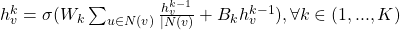where σ is non-linearity (e.g., ReLU), hvk-1 is previous layer embedding of v, sum (after Wk) is average v-th neghbour’s previous layer embeddings.

Embedding after K layers of neighborhood aggregation: zv = hvK.

We can feed these embeddings into any loss function and run stochastic gradient descent to train the weight parameters. We can train in two ways:

1. Unsupervised manner: use only the graph structure.
• “Similar” nodes have similar embeddings.
• Unsupervised loss function can be anything from random walks (node2vec, DeepWalk, struc2vec), graph factorization, node proximity in the graph.
2. Supervised manner: directly train the model for a supervised task (e.g., node classification):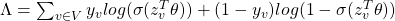where zvT is encoder output (node embedding), yv is node class label, θ is classigication weights.

Supervised training steps:

1. Define a neighborhood aggregation function
2. Define a loss function on the embeddings
3. Train on a set of nodes, i.e., a batch of compute graphs
4. Generate embeddings for nodes as needed

The same aggregation parameters are shared for all nodes: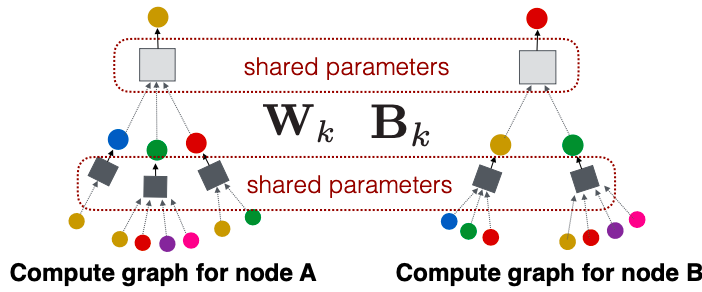The number of model parameters is sublinear in |V| and we can generalize to unseen nodes.

The approach has inductive capability (generate embeddings for unseen nodes or graphs).

###### Graph Convolutional Networks and GraphSAGE

Can we do better than aggregating the neighbor messages by taking their (weighted) average (formula for hvk above)?

Variants

• Mean: take a weighted average of neighbors: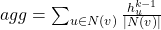• Pool: Transform neighbor vectors and apply symmetric vector function (is element-wise mean/max):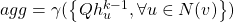• LSTM: Apply LSTM to reshuffled of neighbors: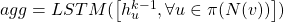Graph convolutional networks average neighborhood information and stack neural networks. Graph convolutional operator aggregates messages across neighborhoods, N(v).

avu = 1/|N(v)| is the weighting factor (importance) of node u’s message to node v. Thus, avu  is defined explicitly based on the structural properties of the graph. All neighbors uN(v) are equally important to node v.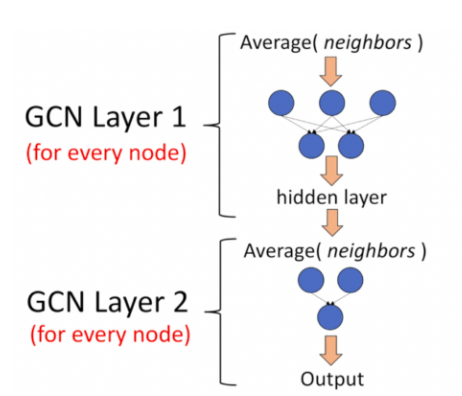Example of 2-layer GCN: The output of the first layer is the input of the second layer. Again, note that the neural network in GCN is simply a fully connected layer (https://www.topbots.com/graph-convolutional-networks/)

GraphSAGE uses generalized neighborhood aggregation:

###### Graph Attention Networks

Idea: Compute embedding hvk of each node in the graph following an attention strategy:

• Nodes attend over their neighborhoods’ message.
• Implicitly specifying different weights to different nodes in a neighborhood.

Let avu be computed as a byproduct of an attention mechanism a. Let a compute attention coefficients evu across pairs of nodes u, v based on their messages: evu = a(Wkhuk-1,Wkhvk-1). evu indicates the importance of node u’s message to node v. Then normalize coefficients using the softmax function in order to be comparable across different neighborhoods: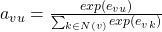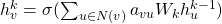Form of attention mechanism a:

• The approach is agnostic to the choice of a
• E.g., use a simple single-layer neural network.
• a can have parameters, which need to be estimates.
• Parameters of a are trained jointly:
• Learn the parameters together with weight matrices (i.e., other parameter of the neural net) in an end-to-end fashion

Paper by Velickovic et al. introduced multi-head attention to stabilize the learning process of attention mechanism:

• Attention operations in a given layer are independently replicated R times (each replica with different parameters). Outputs are aggregated (by concatenating or adding).
• Key benefit: allows for (implicitly) specifying different importance values (avu) to different neighbors.
• Computationally efficient: computation of attentional coefficients can be parallelized across all edges of the graph, aggregation may be parallelized across all nodes.
• Storage efficient: sparse matrix operations do not require more than O(V+E) entries to be stored; fixed number of parameters, irrespective of graph size.
• Trivially localized: only attends over local network neighborhoods.
• Inductive capability: shared edge-wise mechanism, it does not depend on the global graph structure.
###### Example application: PinSage

Pinterest graph has 2B pins, 1B boards, 20B edges and it is dynamic. PinSage graph convolutional network:

• Goal: generate embeddings for nodes (e.g., Pins/images) in a web-scale Pinterest graph containing billions of objects.
• Key Idea: Borrow information from nearby nodes. E.g., bed rail Pin might look like a garden fence, but gates and beds are rarely adjacent in the graph.
• Pin embeddings are essential to various tasks like recommendation of Pins, classification, clustering, ranking.
• Challenges: large network and rich image/text features. How to scale the training as well as inference of node embeddings to graphs with billions of nodes and tens of billions of edges?
• To learn with resolution of 100 vs. 3B, they use harder and harder negative samples – include more and more hard negative samples for each epoch.

Key innovations:

1. On-the-fly graph convolutions:
• Sample the neighborhood around a node and dynamically construct a computation graph.
• Perform a localized graph convolution around a particular node.
• Does not need the entire graph during training.
2. Constructing convolutions via random walks:
• Performing convolutions on full neighborhoods is infeasible.
• Importance pooling to select the set of neighbors of a node to convolve over: define importance-based neighborhoods by simulating random walks and selecting the neighbors with the highest visit counts.
3.  Efficient MapReduce inference:
• Bottom-up aggregation of node embeddings lends itself to MapReduce. Decompose each aggregation step across all nodes into three operations in MapReduce, i.e., map, join, and reduce.

##### General tips working with GNN
• Data preprocessing is important:
• Use renormalization tricks.
• Variance-scaled initialization.
• Network data whitening.
• ADAM optimizer: naturally takes care of decaying the learning rate.
• ReLU activation function often works really well.
• No activation function at your output layer: easy mistake if you build layers with a shared function.
• Include bias term in every layer.
• GCN layer of size 64 or 128 is already plenty.

# Lecture 9 – Graph Neural Networks: Hands-on Session

Video

Colab Notebook has everything to get hands on with PyTorch Geometric. Check it out!

# Lecture 10 – Deep Generative Models for Graphs

In precious lectures, we covered graph encoders where outputs are graph embeddings. This lecture covers the opposite aspect – graph decoders where outputs are graph structures.

###### Problem of Graph Generation

Why is it important to generate realistic graphs (or synthetic graph given a large real graph)?

• Generation: gives insight into the graph formation process.
• Anomaly detection: abnormal behavior, evolution.
• Predictions: predicting future from the past.
• Simulations of novel graph structures.
• Graph completion: many graphs are partially observed.
• “What if” scenarios.

1. Realistic graph generation: generate graphs that are similar to a given set of graphs [Focus of this lecture].
2. Goal-directed graph generation: generate graphs that optimize given objectives/constraints (drug molecule generation/optimization). Examples: discover highly drug-like molecules, complete an existing molecule to optimize a desired property.

Why Graph Generation tasks are hard:

• Large and variable output space (for n nodes we need to generate n*n values; graph size (nodes, edges) varies).
• Non-unique representations (n-node graph can be represented in n! ways; hard to compute/optimize objective functions (e.g., reconstruction of an error)).
• Complex dependencies: edge formation has long-range dependencies (existence of an edge may depend on the entire graph).
###### Graph generative models

Setup: we want to learn a generative model from a set of data points (i.e., graphs) {xi}; pDATA(x) is the data distribution, which is never known to us, but we have sampled xi ~ pDATA(x). pMODEL(x,θ) is the model, parametrized by θ, that we use to approximate pDATA(x).

Goal:

1. Make pMODEL(x,θ) close to pDATA(x) (Key Principle: Maximum Likelihood –  find the model that is most likely to have generated the observed data x).
2. Make sure we can sample from a complex distribution pMODEL(x,θ). The most common approach:
• Sample from a simple noise distribution zi ~ N(0,1).
• Transform the noise zi  via f(⋅): xi = f(zi ; θ). Then xi follows a complex distribution.

To design f(⋅) use Deep Neural Networks, and train it using the data we have.

This lecture is focused on auto-regressive models (predict future behavior based on past behavior). Recap autoregressive models: pMODEL(x,θ) is used for both density estimation and sampling (from the probability density). Then apply chain rule: joint distribution is a product of conditional distributions: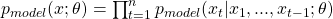In our case: xt will be the t-th action (add node, add edge).

###### GraphRNN

Idea: generating graphs via sequentially adding nodes and edges. Graph G with node ordering π can be uniquely mapped into a sequence of node and edge additions Sπ.

The sequence Sπ has two levels:

• Node-level: at each step, add a new node.
• Edge-level: at each step, add a new edge/edges between existing nodes. For example, step 4 for picture above Sπ4 = (Sπ4,1, Sπ4,2, Sπ431: 0,1,1) means to connect 4&2, 4&3, but not 4&1).

Thus, S is a sequence of sequences: a graph + a node ordering. Node ordering is randomly selected.

We have transformed the graph generation problem into a sequence generation problem. Now we need to model two processes: generate a state for a new node (Node-level sequence) and generate edges for the new node based on its state (Edge-level sequence). We use RNN to model these processes.

GraphRNN has a node-level RNN and an edge-level RNN. Relationship between the two RNNs:

• Node-level RNN generates the initial state for edge-level RNN.
• Edge-level RNN generates edges for the new node, then updates node-level RNN state using generated results.

Setup: State of RNN after time st, input to RNN at time xt, output of RNN at time yt, parameter matrices W, U, V, non-linearity σ(⋅). st = σ(W⋅xt + U⋅ st-1 ), yt = V⋅ st

To initialize s0, x1, use start/end of sequence token (SOS, EOS)- e.g., zero vector. To generate sequences we could let xt+1 = yt. but this model is deterministic. That’s why we use yt = pmodel (xt|x1, …, xt-1; θ). Then xt+1 is a sample from yt: xt+1 ~ yt. In other words, each step of RNN outputs a probability vector; we then sample from the vector, and feed the sample to the next step.

During training process, we use Teacher Forcing principle depicted below: replace input and output by the real sequence.

RNN steps:

1. Assume Node 1 is in the graph. Then add Node 2.
2. Edge RNN predicts how Node 2 connects to Node 1.
3. Edge RNN gets supervisions from ground truth.
4. New edges are used to update Node RNN.
5. Edge RNN predicts how Node 3 connects to Node 2.
6. Edge RNN gets supervisions from ground truth.
7. New edges are used to update Node RNN.
8. Node 4 doesn’t connect to any nodes, stop generation.
9. Backprop through time: All gradients are accumulated across time steps.
10. Replace ground truth by GraphRNN’s own predictions.

GraphRNN has an issue – tractability:

• Any node can connect to any prior node.
• Too many steps for edge generation:
• Need to generate a full adjacency matrix.
• Complex long edge dependencies.

Steps to generate graph below: Add node 1 – Add node 2 – Add node 3 – Connect 3 with 1 and 2 – Add node 4. But then Node 5 may connect to any/all previous nodes.

To limit this complexity, apply Breadth-First Search (BFS) node ordering. Steps with BFS: Add node 1 – Add node 2 – Connect 2 with 1 – Add node 3 – Connect 3 with 1 – Add node 4 – Connect 4 with 2 and 3. Since Node 4 doesn’t connect to Node 1, we know all Node 1’s neighbors have already been traversed. Therefore, Node 5 and the following nodes will never connect to node 1. We only need memory of 2 “steps” rather than n − 1 steps.

Benefits:

• Reduce possible node orderings: from O(n!) to the number of distinct BFS orderings.
• Reduce steps for edge generation (number of previous nodes to look at).

When we want to define similarity metrics for graphs, the challenge is that there is no efficient Graph Isomorphism test that can be applied to any class of graphs. The solution is to use a visual similarity or graph statistics similarity.

###### Application: Drug Discovery

[You et al., NeurIPS 2018]

To learn a model that can generate valid and realistic molecules with high value of a given chemical property, one can use Goal-Directed Graph Generation which:

• Optimize a given objective (High scores), e.g., drug-likeness (black box).
• Obey underlying rules (Valid), e.g., chemical valency rules.
• Are learned from examples (Realistic), e.g., imitating a molecule graph dataset.

Authors of paper present Graph Convolutional Policy Network that combines graph representation and RL. Graph Neural Network captures complex structural information, and enables validity check in each state transition (Valid), Reinforcement learning optimizes intermediate/final rewards (High scores) and adversarial training imitates examples in given datasets (Realistic).

###### Open problems in graph generation
• Generating graphs in other domains:
• 3D shapes, point clouds, scene graphs, etc.
• Scale up to large graphs:
• Hierarchical action space, allowing high level action like adding a structure at a time.
• Other applications: Anomaly detection.
• Use generative models to estimate probability of real graphs vs. fake graphs.

# Lecture 11 – Link Analysis: PageRank

The lecture covers analysis of the Web as a graph. Web pages are considered as nodes, hyperlinks are as edges. In the early days of the Web links were navigational. Today many links are transactional (used to navigate not from page to page, but to post, comment, like, buy, …).

The Web is directed graph since links direct from source to destination pages. For all nodes we can define IN-set (what other nodes can reach v?) and OUT-set (given node v, what nodes can v reach?).

Generally, there are two types of directed graphs:

1. Strongly connected: any node can reach any node via a directed path.
2. Directed Acyclic Graph (DAG) has no cycles: if u can reach v, then v cannot reach u.

Any directed graph (the Web) can be expressed in terms of these two types.

A Strongly Connected Component (SCC) is a set of nodes S so that every pair of nodes in S can reach each other and there is no larger set containing S with this property.

Every directed graph is a DAG on its SCCs:

• SCCs partitions the nodes of G (that is, each node is in exactly one SCC).
•  If we build a graph G’ whose nodes are SCCs, and with an edge between nodes of G’ if there is an edge between corresponding SCCs in G, then G’ is a DAG.

Broder et al.: Altavista web crawl (Oct ’99): took a large snapshot of the Web (203 million URLS and 1.5 billion links) to understand how its SCCs “fit together” as a DAG.

Authors computed IN(v) and OUT(v) by starting at random nodes. The BFS either visits many nodes or very few as seen on the plot below:

Based on IN and OUT of a random node v they found Out(v) ≈ 100 million (50% nodes), In(v) ≈ 100 million (50% nodes), largest SCC: 56 million (28% nodes). It shows that the web has so called “Bowtie” structure:

###### PageRank

There is a large diversity in the web-graph node connectivity -> all web pages are not equally “important”.

The main idea: page is more important if it has more links. They consider in-links as votes. A “vote” (link) from an important page is worth more:

• Each link’s vote is proportional to the importance of its source page.
• If page i with importance ri has di out-links, each link gets ri / di votes.
• Page j’s own importance rj is the sum of the votes on its in-links.

rj = ri /3 + rk/4. Define a “rank” rj for node j (di  is out-degree of node i):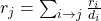If we represent each node’s rank in this way, we get system of linear equations. To solve it, use matrix Formulation.

Let page j have dj out-links. If node j is directed to i, then stochastic adjacency matrix Mij = 1 / dj . Its columns sum to 1. Define rank vector r – an entry per page: ri is the importance score of page i, ∑ri = 1. Then the flow equation is r = M ⋅ r.

###### Random Walk Interpretation

Imagine a random web surfer. At any time t, surfer is on some page i. At time t + 1, the surfer follows an out-link from i uniformly at random. Ends up on some page j linked from i. Process repeats indefinitely. Then p(t) is a probability distribution over pages, it’s a vector whose ith coordinate is the probability that the surfer is at page i at time t.

To identify where the surfer is at time t+1, follow a link uniformly at random p(t+1) = M ⋅ p(t). Suppose the random walk reaches a state  p(t+1) = M ⋅ p(t) = p(t). Then p(t) is stationary distribution of a random walk. Our original rank vector r satisfies r = Mr. So, r is a stationary distribution for the random walk.

As flow equations is r = M ⋅ r, r is an eigenvector of the stochastic web matrix M. But Starting from any vector u, the limit M(M(…(M(Mu))) is the long-term distribution of the surfers. With math we derive that limiting distribution is the principal eigenvector of M – PageRank. Note: If r is the limit of M(M(…(M(Mu)), then r satisfies the equation r = Mr, so r is an eigenvector of M with eigenvalue 1. Knowing that, we can now efficiently solve for r with the Power iteration method.

Power iteration is a simple iterative scheme:

• Initialize: r(0) = [1/N,….,1/N]T
• Iterate:  r(t+1) = M ·  r(t)
• Stop when |r(t+1) – r(t)|1 < ε (Note that |x|1 = Σ|xi| is the L1 norm, but we can use any other vector norm, e.g., Euclidean). About 50 iterations is sufficient to estimate the limiting solution.
###### How to solve PageRank

Given a web graph with n nodes, where the nodes are pages and edges are hyperlinks:

• Assign each node an initial page rank.
• Repeat until convergence (Σ |r(t+1) – r(t)|1 < ε).

Calculate the page rank of each node (di …. out-degree of node i):PageRank solution poses 3 questions: Does this converge? Does it converge to what we want? Are results reasonable?

PageRank has some problems:

1. Some pages are dead ends (have no out-links, right part of web “bowtie”): such pages cause importance to “leak out”.
2. Spider traps (all out-links are within the group, left part of web “bowtie”): eventually spider traps absorb all importance.

Solution for spider traps: at each time step, the random surfer has two options:

Common values for β are in the range 0.8 to 0.9. Surfer will teleport out of spider trap within a few time steps.

Why are dead-ends and spider traps a problem and why do teleports solve the problem?

• Spider-traps are not a problem, but with traps PageRank scores are not what we want.
• Solution: never get stuck in a spider trap by teleporting out of it in a finite number of steps.
• Dead-ends are a problem: the matrix is not column stochastic so our initial assumptions are not met.
• Solution: make matrix column stochastic by always teleporting when there is nowhere else to go.

This leads to PageRank equation [Brin-Page, 98]: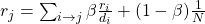This formulation assumes that M has no dead ends. We can either preprocess matrix M to remove all dead ends or explicitly follow random teleport links with probability 1.0 from dead-ends. (di … out-degree of node i).

The Google Matrix A becomes as: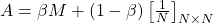[1/N]NxN…N by N matrix where all entries are 1/N.

We have a recursive problem: r = A ⋅ r and the Power method still works.

###### Computing Pagerank

The key step is matrix-vector multiplication: rnew = A · rold. Easy if we have enough main memory to hold each of them. With 1 bln pages, matrix A will have N2 entries and 1018 is a large number.

But we can rearrange the PageRank equation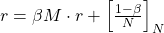where [(1-β)/N]N is a vector with all N entries (1-β)/N.

M is a sparse matrix (with no dead-ends):10 links per node, approx 10N entries. So in each iteration, we need to: compute rnew = β M · rold and add a constant value (1-β)/N to each entry in rnew. Note if M contains dead-ends then ∑rj new < 1 and we also have to renormalize rnew so that it sums to 1.

###### Random Walk with Restarts

Idea: every node has some importance; importance gets evenly split among all edges and pushed to the neighbors. Given a set of QUERY_NODES, we simulate a random walk:

• Make a step to a random neighbor and record the visit (visit count).
• With probability ALPHA, restart the walk at one of the QUERY_NODES.
• The nodes with the highest visit count have highest proximity to the QUERY_NODES.

The benefits of this approach is that it considers: multiple connections, multiple paths, direct and indirect connections, degree of the node.

PS: Other lecture notes you can find here: 13, 4.

## Machine Learning with Graphs: lecture notes, part 1/4

Recently, I finished the Stanford course CS224W Machine Learning with Graphs. In the following series of blog posts, I share my notes which I took watching lectures. I hope it gives you a quick sneak peek overview of how ML applied for graphs. The rest of the blog posts you can find here: 2, 3, 4.

NB: I don’t have proofs and derivations of theorems here, check out original sources if you are interested.

# Lecture 1 – Intro to graph theory

Slides, Video

The lecture gives an overview of basic terms and definitions used in graph theory. Why should you learn graphs? The impact! The impact of graphs application is proven for Social networking, Drug design, AI reasoning.

My favourite example of applications is the Facebook social graph which shows that all users have only 4-degrees of separation:

Another interesting example of application: predict side-effects of drugs (when patients take multiple drugs at the same time, what are the potential side effects? It represents a link prediction task).

What are the ways to analyze networks:

1. Node classification (Predict the type/color of a given node)
3. Identify densely linked clusters of nodes (Community detection)
4. Network similarity (Measure similarity of two nodes/networks)

Key definitions:

• A network is a collection of objects (nodes) where some pairs of objects are connected by links (edges).
• Types of networks: Directed vs. undirected, weighted vs. unweighted, connected vs. disconnected
• Degree of node i is the number of edges adjacent to node i.
• The maximum number of edges on N nodes (for undirected graph) is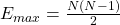• Complete graph is an undirected graph with the number of edges E = Emax, and its average degree is N-1.
• Bipartite graph is a graph whose nodes can be divided into two disjoint sets U and V such that every link connects a node in U to one in V; that is, U and V are independent sets (restaurants-eaters for food delivery, riders-drivers for ride-hailing).

# Lecture 2 – Properties of networks. Random Graphs

Slides, Video

Metrics to measure network:

• Degree distribution, P(k): Probability that a randomly chosen node has degree k

Nk = # nodes with degree k.

• Path length, h: a length of the sequence of nodes in which each node is linked to the next one (path can intersect itself).
• Distance (shortest path, geodesic) between a pair of nodes is defined as the number of edges along the shortest path connecting the nodes. If the two nodes are not connected, the distance is usually defined as infinite (or zero).
• Diameter: maximum (shortest path) distance between any pair of nodes in a graph.
• Clustering coefficient, Ci: describes how connected are i’s neighbors to each other.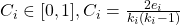where ei is the number of edges between neighbors of node i.

• Average clustering coefficient: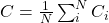• Connected components, s: a subgraph in which each pair of nodes is connected with each other via a path (for real-life graphs, they usually calculate metrics for the largest connected components disregarding unconnected nodes).
###### Erdös-Renyi Random Graphs:
• Gnp: undirected graph on n nodes where each edge (u,v) appears i.i.d. with probability p.
• Degree distribution of Gnp is binomial.
• Average path length: O(log n).
• Average clustering coefficient: k / n.
• Largest connected component: exists if k > 1.

Comparing metrics of random graph and real life graphs, real networks are not random. While Gnp is wrong, it will turn out to be extremely useful!

Real graphs have high clustering but diameter is also high. How can we have both in a model? Answer in Small-World Model [Watts-Strogatz ‘98].

Small-World Model introduces randomness (“shortcuts”): first, add/remove edges to create shortcuts to join remote parts of the lattice; then for each edge, with probability p, move the other endpoint to a random node.

Kronecker graphs: a recursive model of network structure obtained by growing a sequence of graphs by iterating the Kronecker product over the initiator matrix. It may be illustrated in the following way:

Random graphs are far away from real ones, but real and Kronecker networks are very close.

# Recitation 1 – Introduction to snap.py

Slides

Stanford Network Analysis Platform (SNAP) is a general purpose, high-performance system for analysis and manipulation of large networks (http://snap.stanford.edu). SNAP software includes Snap.py for Python, SNAP C++ and SNAP datasets (over 70 network datasets can be found at https://snap.stanford.edu/data/).

Useful resources for plotting graph properties:

I will not go into details here, better to get hands on right away with the original snappy tutorial at https://snap.stanford.edu/snappy/doc/tutorial/index-tut.html.

# Lecture 3 – Motifs and Structural Roles in Networks

Slides, Video

Subnetworks, or subgraphs, are the building blocks of networks, they have the power to characterize and discriminate networks.

Network motifs are:

Motifs help us understand how networks work, predict operation and reaction of the network in a given situation.

Motifs can overlap each other in a network.

Examples of motifs:

Significance of motifs: Motifs are overrepresented in a network when compared to randomized networks. Significance is defined as:where Nireal is # of subgraphs of type i in network Greal and Nireal is # of subgraphs of type i in network Grand.

• Zi captures statistical significance of motif i.
• Generally, larger networks display higher Z-scores.
• To calculate Zi, one counts subgraphs i in Greal, then counts subgraphs i in Grand with a configuration model which has the same #(nodes), #(edges) and #(degree distribution) as Greal.
###### Graphlets

Graphlets are connected non-isomorphic subgraphs (induced subgraphs of any frequency). Graphlets differ from network motifs, since they must be induced subgraphs, whereas motifs are partial subgraphs. An induced subgraph must contain all edges between its nodes that are present in the large network, while a partial subgraph may contain only some of these edges. Moreover, graphlets do not need to be over-represented in the data when compared with randomized networks, while motifs do.

Node-level subgraph metrics:

• Graphlet degree vector counts #(graphlets) that a node touches.
• Graphlet Degree Vector (GDV) counts #(graphlets) that a node touches.

Graphlet degree vector provides a measure of a node’s local network topology: comparing vectors of two nodes provides a highly constraining measure of local topological similarity between them.

Finding size-k motifs/graphlets requires solving two challenges:

• Enumerating all size-k connected subgraphs
• Counting # of occurrences of each subgraph type via graph isomorphisms test.

Just knowing if a certain subgraph exists in a graph is a hard computational problem!

• Subgraph isomorphism is NP-complete.
• Computation time grows exponentially as the size of the motif/graphlet increases.
• Feasible motif size is usually small (3 to 8).

Algorithms for counting subgaphs:

• Exact subgraph enumeration (ESU) [Wernicke 2006] (explained in the lecture, I will not cover it here).
• Kavosh [Kashani et al. 2009].
• Subgraph sampling [Kashtan et al. 2004].

Structural roles in networks

Role is a collection of nodes which have similar positions in a network

• Roles are based on the similarity of ties between subsets of nodes.
• In contrast to groups/communities, nodes with the same role need not be in direct, or even indirect interaction with each other.
• Examples: Roles of species in ecosystems, roles of individuals in companies.
• Structural equivalence: Nodes are structurally equivalent if they have the same relationships to all other nodes.

Examples when we need roles in networks:

• Role query: identify individuals with similar behavior to a known target.
• Role outliers: identify individuals with unusual behavior.
• Role dynamics: identify unusual changes in behavior.

Structural role discovery method RoIX:

• Turn network connectivity into structural features with Recursive feature Extraction [Henderson, et al. 2011a]. Main idea is to aggregate features of a node (degree, mean weight, # of edges in egonet, mean clustering coefficient of neighbors, etc) and use them to generate new recursive features (e.g., mean value of “unweighted degree” feature between all neighbors of a node). Recursive features show what kinds of nodes the node itself is connected to.
• Cluster nodes based on extracted features. RolX uses non negative matrix factorization for clustering, MDL for model selection, and KL divergence to measure likelihood.

# Lecture 4 – Community Structure in Networks

Slides, Video

The lecture shows methods for community detection problems (nodes clustering).

Background example from Granovetter work, 1960s: people often find out about new jobs through acquaintances rather than close friends. This is surprising: one would expect your friends to help you out more than casual acquaintances.

Why is it that acquaintances are most helpful?

• Long-range edges (socially weak, spanning different parts of the network) allow you to gather information from different parts of the network and get a job.
• Structurally embedded edges (socially strong) are heavily redundant in terms of information access.

From this observation we find one type of structural role –  Triadic Closure: if two people in a network have a friend in common, then there is an increased likelihood they will become friends themselves.

Triadic closure has high clustering coefficient. Reasons: if A and B have a friend C in common, then:

• A is more likely to meet B (since they both spend time with C).
• A and B trust each other (since they have a friend in common).
• C has incentive to bring A and B together (since it is hard for C to maintain two disjoint relationships).

Granovetter’s theory suggests that networks are composed of tightly connected sets of nodes and leads to the following conceptual picture of networks:

###### Network communities

Network communities are sets of nodes with lots of internal connections and few external ones (to the rest of the network).

Modularity is a measure of how well a network is partitioned into communities: the fraction of the edges that fall within the given groups minus the expected fraction if edges were distributed at random.

Given a partitioning of the network into groups disjoint s: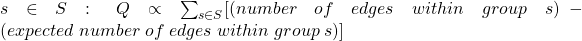Modularity values take range [−1,1]. It is positive if the number of edges within groups exceeds the expected number. Values greater than 0.3-0.7 means significant community structure.

To define the expected number of edges, we need a Null model. After derivations (can be found in slides), modularity is defined as: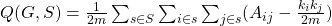• Aij represents the edge weight between nodes i and j;
• ki and kj are the sum of the weights of the edges attached to nodes i and j;
• 2m is the sum of all the edges weights in the graph.
###### Louvian algorithm

Louvian algorithm is a greedy algorithm for community detection with O(n log(n)) run time.

• Supports weighted graphs.
• Provides hierarchical communities.
• Widely utilized to study large networks because it is fast, has rapid convergence and high modularity output (i.e., “better communities”).

Each pass of Louvain algorithm is made of 2 phases:

Phase 1 – Partitioning:

• Modularity is optimized by allowing only local changes to node-communities memberships;
• For each node i, compute the modularity delta (∆Q) when putting node i into the community of some neighbor j
• Move i to a community of node j that yields the largest gain in ∆Q.

Phase 2 – Restructuring:

• identified communities are aggregated into super-nodes to build a new network;
• communities obtained in the first phase are contracted into super-nodes, and the network is created accordingly: super-nodes are connected if there is at least one edge between the nodes of the corresponding communities;
• the weight of the edge between the two supernodes is the sum of the weights from all edges between their corresponding communities;
• goto Phase 1 to run it on the super-node network.

The passes are repeated iteratively until no increase of modularity is possible.

###### Detecting overlapping communities: BigCLAM

BigCLAM is a model-based community detection algorithm that can detect densely overlapping, hierarchically nested as well as non-overlapping communities in massive networks (more details and all proofs are in Yang, Leskovec “Overlapping Community Detection at Scale: A Nonnegative Matrix Factorization Approach”, 2013).

In BigCLAM communities arise due to shared community affiliations of nodes. It explicitly models the affiliation strength of each node to each community. Model assigns each node-community pair a nonnegative latent factor which represents the degree of membership of a node to the community. It then models the probability of an edge between a pair of nodes in the network as a function of the shared community affiliations.

Step 1: Define a generative model for graphs that is based on node community affiliations –  Community Affiliation Graph Model (AGM). Model parameters are Nodes V, Communities C, Memberships M. Each community c has a single probability pc.

Step 2: Given graph G, make the assumption that G was generated by AGM. Find the best AGM that could have generated G. This way we discover communities.

# Lecture 5 – Spectral Clustering

Slides, Video

NB: these notes skip lots of mathematical proofs, all derivations can be found in slides themselves.

Three basic stages of spectral clustering:

1. Pre-processing: construct a matrix representation of the graph.
2. Decomposition: compute eigenvalues and eigenvectors of the matrix; map each point to a lower-dimensional representation based on one or more eigenvectors.
3. Grouping: assign points to two or more clusters, based on the new representation.

Lecture covers them one by one starting with graph partitioning.

###### Graph partitioning

Bi-partitioning task is a division of vertices into two disjoint groups A and B. Good partitioning will maximize the number of within-group connections and minimize the number of between-group connections. To achieve this, define edge cut: a set of edges with one endpoint in each group:Graph cut criterion:

• Minimum-cut: minimize weight of connections between groups. Problems: it only considers external cluster connections and does not consider internal cluster connectivity.
• Conductance: connectivity between groups relative to the density of each group. It produces more balanced partitions, but computing the best conductance cut is NP-hard.

Conductance  φ(A,B) = cut(A, B) / min(total weighted degree of the nodes in A or in B)

To efficiently find good partitioning, they use Spectral Graph Theory.

Spectral Graph Theory analyze the “spectrum” of matrix representing G: Eigenvectors x(i) of a graph, ordered by the magnitude (strength) of their corresponding eigenvalues λi.

Λ = {λ1,λ2,…,λn}, λ1≤ λ2≤ …≤ λn (λi are sorted in ascending (not descending) order).

If A is adjacency matrix of undirected G (aij=1 if (i,j) is an edge, else 0), then Ax = λx.

What is the meaning of Ax? It is a sum of labels xj of neighbors of i. It is better seen if represented in the form as below:

Examples of eigenvalues for different graphs:

For d-regular graph (where all nodes have degree d) if try x=(1,1,1,…1):

Ax = (d,d,..,d) = λx -> λ = d. This d is the largest eigenvalue of d-regular graph (proof is in slides).

An N x N matrix can have up to n eigenpairs.

Matrix Representation:

• Adjacency matrix (A): A = [aij], aij = 1 if edge exists between node i and j (0-s on diagonal). It is a symmetric matrix, has n real eigenvalues; eigenvectors are real-valued and orthogonal.
• Degree matrix (D): D=[dij],dijis a degree of node i.
• Laplacian matrix (L): L = D-A. Trivial eigenpair is with x=(1,…,1) -> Lx = 0 and so λ = λ1 = 0.

So far, we found λ and λ1. From theorems, they derive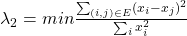(all labelings of node i so that sum(xi) = 0, meaning to assign values xi to nodes i such that few edges cross 0  (xi and xj to subtract each other as shown on the picture below).

From theorems and profs, we go to the Cheeger inequality: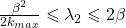where kmax is the maximum node degree in the graph and β is conductance of the cut (A, B).

β = (# edges from A to B) / |A|. This is the approximation guarantee of the spectral clustering: spectral finds a cut that has at most twice the conductance as the optimal one of conductance β.

To summarize 3 steps of spectral clustering:

1. Pre-processing: build Laplacian matrix L of the graph.
2. Decomposition: find eigenvalues λ and eigenvectors x of the matrix L and map vertices to corresponding components of x2.
3. Grouping: sort components of reduced 1-dimensional vector and identify clusters by splitting the sorted vector in two.

How to choose a splitting point?

• Naïve approaches: split at 0 or median value.
• More expensive approaches: attempt to minimize normalized cut in 1-dimension (sweep over ordering of nodes induced by the eigenvector).
###### K-way spectral clustering:

Recursive bi-partitioning [Hagen et al., ’92] is the method of recursively applying bi-partitioning algorithm in a hierarchical divisive manner. It has disadvantages: inefficient and unstable.

More preferred way:

Cluster multiple eigenvectors [Shi-Malik, ’00]: build a reduced space from multiple eigenvectors. Each node is now represented by k numbers; then we cluster (apply k-means) the nodes based on their k-dimensional representation.

To select k, use eigengap: the difference between two consecutive eigenvalues (the most stable clustering is generally given by the value k that maximizes eigengap).

###### Motif-based spectral clustering

Different motifs will give different modular structures. Spectral clustering for nodes generalizes for motifs as well.

Similarly to edge cuts, volume and conductance, for motifs:

volM(S) = #(motif endpoints in S), φ(S) = #(motifs cut) / volM(S).

Motif Spectral Clustering works as follows:

• Input: Graph G and motif M.
• Using G form a new weighted graph W(M).
• Apply spectral clustering on W(M).
• Output the clusters.

Resulting clusters will obtain near optimal motif conductance.

The same 3 steps as before:

1. Preprocessing: given motif M. Form weighted graph W(M).

Wij(M)=# times edge (i,j) participates in the motif M.

1. Apply spectral clustering: Compute Fiedler vector x associated with λ2 of the Laplacian of L(M).

L(M) = D(M) – W(M) where degree matrix Dij(M) = sum(Wij(M)). Compute L(M)x = λ2x. Use x to identify communities.

1. Sort nodes by their values in x: x1, x2, …xn. Let Sr = {x1, x2, …xn} and compute the motif conductance of each Sr.

At the end of the lecture, there are two examples of how motif-based clustering is used for food webs and gene regulatory networks.

There exist other methods for clustering:

PS: Other lecture notes you can find here: 23, 4.

## How many connections are there between two network nodes: the story of searches and blog posts

I am really excited about graphs and networks. I get used to the idea that everything and everyone in the world is linked to each other within a low number of connections, but still, I couldn’t imagine that you can have so many connections to one node in the network accumulated from different sources.

One day my friend noticed that my voice is different when I speak different languages. My voice in Russian has a low pitch, but when speaking in English, it sounds higher. This discovery surprised me: I’ve never noticed it by myself, so I started to think about why it happens. My first assumption was that as English is not my native language, sometimes I can be less confident in words (and especially in my pronunciation with Russian accent), so it increases the voice pitch (aka frequency). Then I started to google and out of many reddit/quora threads, I stumbled upon a blog post with a rich data analysis of voices (which additionally fascinated me as I love data).

The blog post Language pitch by Erik Bernhardsson describes the substantial pitch differences between languages and makes the assumption that “there might be a “natural” pitch of each language that is optimized for pronouncing words with the least amount of effort”. Out of all articles I found on this topic, I like this one the most, because it described the idea simply, showed conclusions based on data, and it also has details about how the analysis was performed. It sparked my interest and I wanted to see what else I can find written by this author.

[Small step back. The same day in the morning, my friend showed me Annoy library which can be used for building recommendation systems. Also, I got to know that the library was built by a Spotify engineer during their Hack Week.]

So, I opened the “About” part on Erik’ website and what I saw: “I was at Spotify for 6 years … I also open sourced a couple of tools, including … Annoy – a library to find approximate nearest neighbors in high dimensional spaces.” Ok, it seems I already had a connection to the author and now it is one more (2 in total). What else can I find on his website?

I started to skim through the Top posts and I found: “How to hire smarter than the market” – a friend cited it to me dozens of times when we talked about working in tech (counting 3 connections so far). When reading a post about simulating buffet lines, I checked a link to the convoys library which seemed familiar to me. And yeah, the github readme directed to “Modeling conversion rates and saving millions of dollars using Kaplan-Meier and gamma distributions“ – the article I read in Data Elixir mailing list in August 2019. A similar story is with the post “Why software projects take longer than you think: a statistical model“ from DataScienceWeekly mailing list from August 2019 (counting 5 connections now). One more post  about conversion rates I found and shared with colleagues about a year ago when researching user retention topics (6 connections in total).

At this point, I stopped counting and just enjoyed reading Erik’s writings. What excites me is that he applies modeling and data approach to different ranges of problems:  from hiring to career to management in tech, etc. That is really cool and I advise you to check his posts.

And remember, graphs are everywhere!

## Bootstrapping Confidence Intervals: the basics

If you are a data scientist, data analysts, product manager in a data-driven company, you experiment with your product a lot, and usually you do lots of AB tests.

Let’s assume you have an AB test with control and treatment groups. When analysing results, you compare the average number of orders/visits/views (or any other metric) in test groups. Obviously, you can calculate the mean of a metric, you can even say using t-test and p-value if there is statistically significant difference between two means. But usually, they say don’t look only at p-values, look at confidence intervals.

In this blog post, we discover how to calculate confidence intervals using bootstrapping. Bootstrapping is a cool method to estimate confidence intervals because it does not rely on any assumption of data distribution (compared to popular Welch t-test, for example) and it is quite easy to implement by yourself (I share a link to my implementation below).

## Bootstrap definition and principle

The bootstrap is a method for estimating standard errors and computing confidence intervals. Bootstrapping started in 1970th by Bradley Efron; it has already existed for more than 40 years, so many different types and methods of bootstrapping were developed since then.

But despite of many variations, all  bootstrap steps look as follows:

1. Obtain data sample x1,x2,…,xn drawn from a distribution F.
2. Define u – statistic computed from the sample (mean, median, etc).
3. Sample x*1, x*2, . . . , x*n with replacement from the original data sample. Let it be F*  –  the empirical distribution. Repeat n times (n is bootstrap iterations).
4. Compute u* –  the statistic calculated from each resample.

Then the bootstrap principle says that:

1. Empirical distribution from bootstraps is approximately equal to distribution of sample data:  F* ≈ F.
2. The variation of statistic computed from the sample u is well-approximated by the variation of statistic computed from each resample u*. So, computed statistic u* from bootstrapping is a good proxy for statistic of sample data.

As mentioned, bootstrap can be applied for different goals:

• Estimating Confidence intervals
• Hypothesis testing
• Bias elimination

Each topic deserves a separate post and today we concentrate only on bootstrapping confidence intervals.

## Methods for Bootstrapping Confidence Intervals

When I researched the topic, I haven’t found a single source with all bootstraps methods at once, every reference has its own types. Most common ones are presented below:

1. Empirical Bootstrapping

In a nutshell, this method approximates the difference between bootstrapped means and sample mean.

Let’s consider an example (it will continue for other methods as well): estimate the mean μ of the underlying distribution and give an 80% bootstrap confidence interval.

Empirical bootstrapping says:

• For each bootstrap calculate mean x*
• Compute δ* = x* − x for each bootstrap sample (x is mean of original data), sort them from smallest to biggest.
• Choose δ.1 as the 90th percentile, δ.9 as the 10th percentile of sorted list of δ*, which gives an 80% confidence interval of [x−δ.1, x−δ.9].

Wasserman’s “All of Statistics” book provides with the following math notation for empirical bootstrap:

where x is mean of original data and x* is bootstrap means.

2. Percentile bootstrap

Instead of computing the differences δ*, the bootstrap percentile method uses the distribu­tion of the bootstrap sample statistic as a direct approximation of the data sample statistic.

For Percentile bootstrap:

• For each bootstrap calculate mean x*, sort them from smallest to biggest.
• Choose x*.1 as the 10th percentile, x*.9 as the 90th percentile of sorted list of x*, which gives an 80% confidence interval of [x*.1, x*.9].

Or, in other words:

The bootstrap percentile method is quite simple, but it depends on the bootstrap distribution of x* based on a particular sample being a good approximation to the true distribution of x (because of that reason, this source advises not to use percentile bootstrap).

3. Normal bootstrap

Instead of taking percentiles of bootstrapped means, normal bootstrap method calculates confidence intervals for these bootstrapped means.

• For each bootstrap calculate mean x*.
• Calculate 80% confidence intervals for array of x* using for example, Student t-test:

where x* is the sample mean from bootstrap means, se is the standard error of the bootstrap means, z* is critical value (found from the distribution table of normal CDF).

When I compared this method to others, its CI were always very narrow compared to other methods which puzzled me a lot. As I understood, it is because the definition strongly relies on assumption about normal distribution of data.

Some time later, I stumbled upon slightly different wording of this method in Wasserman’s “All of Statistics”. Calculation differs by just one parameter, but it gives a huge difference.

4. Normal interval bootstrap

Normal interval bootstrap repeats all steps of normal bootstrap, but use the following formula for CI:

where se_boot is the bootstrap estimate of the standard error.

This interval is not accurate unless the distribution of x* is close to Normal.

Basically, here formula has no division by squared root of n. I haven’t found explanation why it is done in that way. If you have any ideas, please, share them in comments.

5. Parametric Bootstrap

Parametric bootstrap is very close to empirical approach, the only difference is in the source of the bootstrap sample. For the parametric bootstrap, we generate the bootstrap sample from a parametrized distribu­tion. It is often used for the efficient computation of Bayes posterior distributions, not for experimentation analysis, so I will not go into its details here.

## Comparing methods

As promised earlier, here you can find Python implementation of all methods. The goal was to estimate 95% bootstrap confidence interval for the mean of target metric. I played with bootstrap methods, number of bootstrap samples and sample size of data itself.

The main question I had: which bootstrap method would show more reliable results. First of all, normal bootstrap crearly produces too narrow CI (because of normality assumptions). Other 3 methods are usually close to each other given large enough sample. The advantage of percentile and empirical types is that they provide different intervals from left and right sides (in contrast to normal interval bootstrap). Usually, it is better because it accounts for distribution of data. As you can see on plot below,  intervals for percentile and empirical bootstraps are greeter from the left side – on the side where population mean lies.

Choosing between percentile and empirical methods, I am more inclined towards the percentile approach because of its simplicity. Its results are very close to the empirical method, and at the same time requires one step less in calculations.

Another important question: how many bootstrap samplings to do. It depends on data size. If there are less than 1000 data points, it is reasonable to take bootstrap number no more than twice less data size (if there are 400 samples, use no more than 200 bootstraps – further increase doesn’t provide any improvements). If you have more data, the number of bootstraps between 100 and 500 is sufficient (higher number usually will not increase accuracy of CI).

One critical remark one should remember using bootstrapping is that it can’t improve point estimate, meaning the quality of bootstrapping depends on the quality of collected data. If sample data is biased and doesn’t represent population data well, the same will occur with bootstrap estimates. So, always keep in mind that data collected during experimentation should be a good approximation of the whole population data.

In this blog post, I described and compared basic bootstrap methods. Actually, there exist many more methods (like Poisson, Gaussian, Block, etc). Also, bootstrap is not only about confidence intervals, it is used for estimation of standard error of the median, 75% percentiles, hypothesis testing, etc. All of them are good topics for next blog posts 🙂

## Remote work: tips & tricks

In March 2020, the situation with the worldwide pandemic of Coronavirus COVID-19  spreading in Europe started to rapidly worsen every day. On March, 10, all employees in my company were advised to work from home. On March, 12, Estonia declared the emergency situation in the whole country, and we were not advised, but forced to stay home and all colleagues suddenly switched to remote work.

I worked remotely before, but it was for a couple of days in a row, not for weeks. As for my team, it was usually a few people out of office, not the whole team distributed across many places. This sudden switch to working from home (WFH) was not very easy, but manageable, and now after a month, I want to share tips & tricks for remote work, how to stay productive and keep connection with colleagues.

## Tip 1 – Set up your workplace

Your working place affects your work a lot. Be sure to have a comfortable chair and desk, monitors if you need ones, good headphones for calls. Working from bed may seem attractive, but your body got used to sleeping there and just having a laptop on your lap will not force it to work: you will feel very distracted and sleepy. Ideally, try to set up a special place where you just work and do nothing else, it helps to switch your brain from working/not working state faster. If you don’t have a desk and office chair, see if you can buy them or ask if it is possible to take them from your office. Recently, I bought a standing desk (before I had only a dining table at home which I used as a desk) and definitely, my back is very grateful for that (in the office, I used to stand most of the time, and constant sitting at home sometimes is painful for me).

## Tip 2 – Get a routine for starting/ending working day

During the first fully remote week, I started to work at the same time as I did at the office, but I noticed that in the evening, I just worked and worked, continuing finishing tasks lately in the evening. It happened, because there was no need to go home from the office, and there was no conditioning for the brain to switch between activities. It is very important to keep the work-life balance at home office as well. Helpful tool for that is time tracking (for example, I use Toogl app) – it gives you a true estimation of how much time spent on different activities. Also, good advice is to have a walk before and/or after working hours to reset your mind on fresh air and have some physical activity.

## Tip 3 – Find your own most productive day plan

In tech work, concentration is a key and every distraction may ruin it easily. That’s why it is important to find your own way how to handle increased online communication with colleagues and how to schedule daily/weekly calls. Try to split time reserved for meetings and for deep uninterrupted work and let your colleagues know about it. For example, agree on morning or evening calls, depending on when you prefer to talk and to work; midday usually is not the best choice because it breaks day leaving less time for other things. Also, nice to have some days without any meetings to have timeslots only for concentrated work.

Now, as set up and working habits are ready, some thoughts on collaboration with teams in remote mode.

## Tip 4 – Calls with Video

At home office, you may feel alone and disconnected from others. To eliminate this, when having calls, always switch on camera and don’t be afraid to ask colleagues to do the same. It is more relevant for small groups discussion where everybody is involved. First, it is important because people perceive information not only from voice, but from gestures and face mimics as well. Second, camera forces you to be more engaged in conversation, leaving fewer chances for you to start checking slack or email. Notice next time, how you behave and where your attention mostly lies during calls with and without video.

One more thing about calls in general: test in advance your headphones and be sure that your sound doesn’t produce any background noise (many apps have this feature). Make it easier and less painful for others to hear you and understand what you are saying.

## Tip 5 – Over Communicate

Remote work makes communication between people harder. I miss those moments when I went to the office kitchen for a coffee, randomly met people, got to know some news, had spontaneous discussions on a project. But now, try to imagine your channels in slack as this “virtual room”. Try to write more in channels than in direct messages, share more with colleagues – things which you found helpful, interesting articles, recent news about your field (but find a limit not to spam them). If you want to clarify some parts of the task, remember that a 5 minutes call can be more useful than a long written conversation. Don’t be shy to ask for a short call from teammates to ask what you don’t understand or need to clarify – it may be less time consuming for both sides.

## To sum up

After a month of fully remote work, I feel that actually my productivity at home even increased compared to the office. I learned how to have fewer distractions, how to keep work-life balance staying at home and how to communicate with the team fully remotely.

Nobody knows when the situation with COVID-19 will improve and when we will start coming back from self-isolation. But it is a good opportunity to try working from home for somebody who hasn’t done it before. Maybe such a setting would look more appealing and s/he would like to continue remote work after the crisis as well.

But for now, stay healthy and stay home!